# Karnataka SSLC Board (KSEEB) Question Paper for Class 10th Maths 2018 In PDF

## Karnataka SSLC or Class 10 Maths Question Paper 2018 with Solutions – Free Download

KSEEB SSLC Maths question paper 2018 with answers are available at BYJU’S to help the students in verifying their solutions, after solving the SSLC 2018 question paper. They can download the SSLC question paper 2018 Karnataka pdf to practise the questions, as a part of the preparation for the examination. Besides, students can also access the SSLC model question paper 2018-19 to just download the pdf and practise easily. All the recent years, KSEEB SSLC Class 10 Maths question papers are accessible in a downloadable pdf format here. However, KSEEB Maths question papers 2018, will help the students to get a thorough understanding of the pattern of question papers in the examination.

### KSEEB Class 10 Maths Question Paper Solution 2018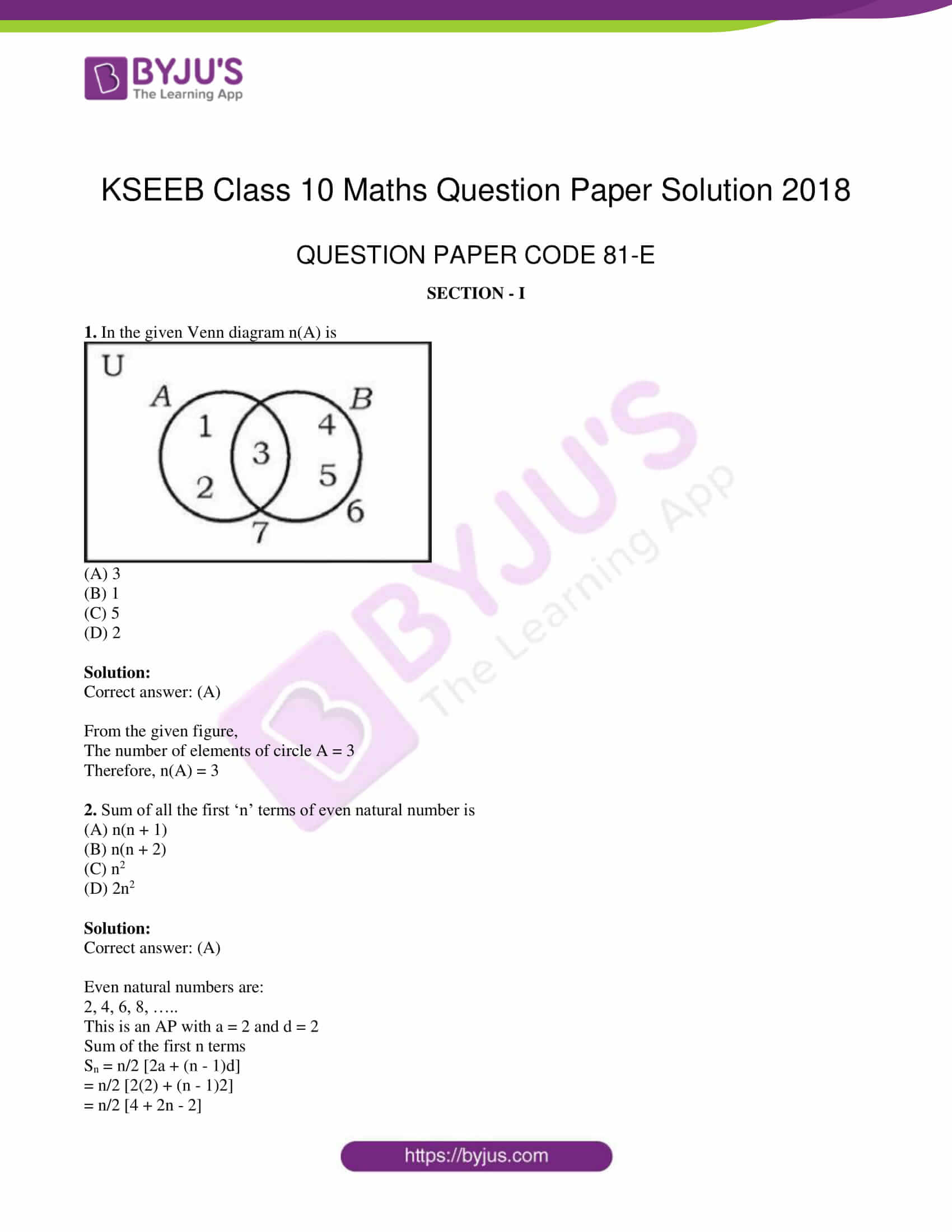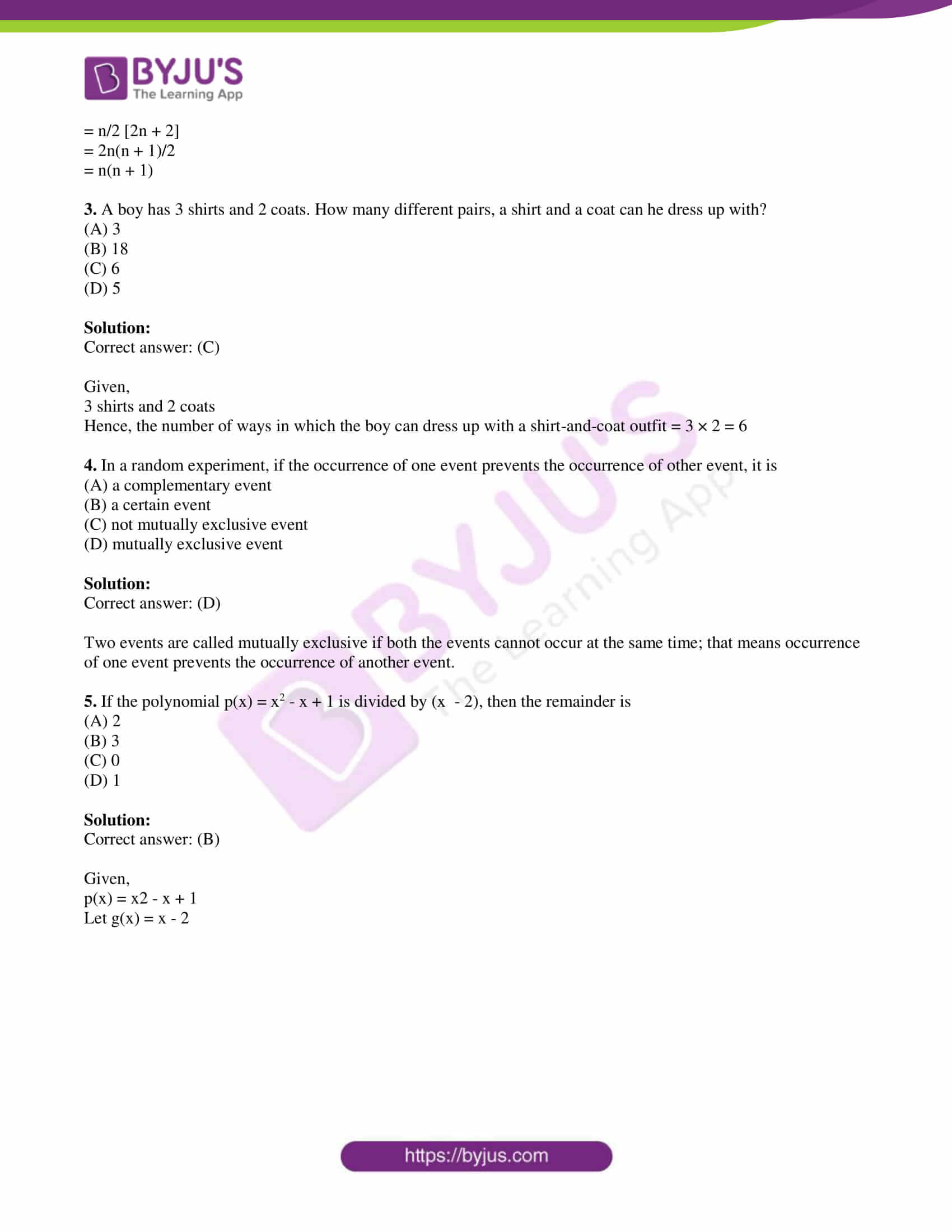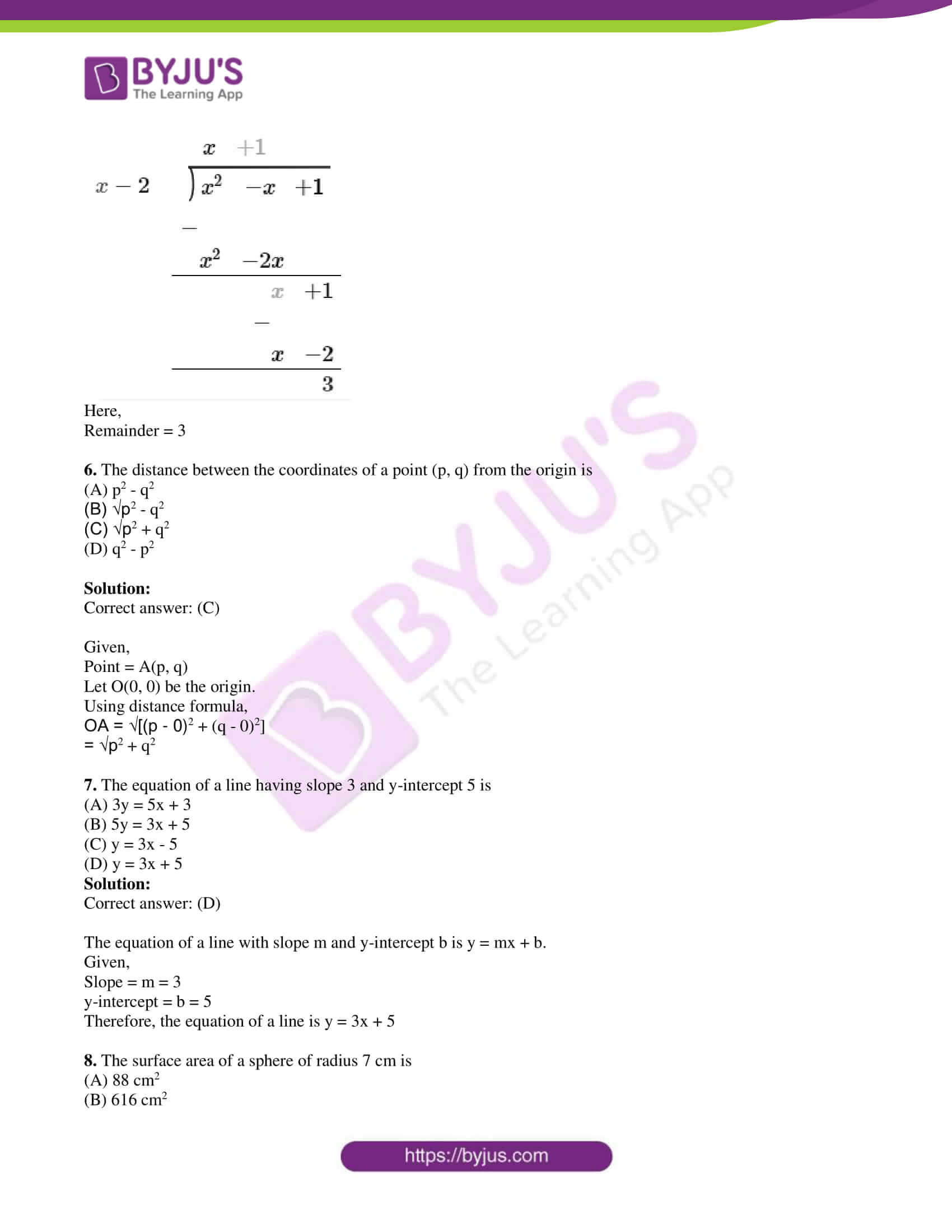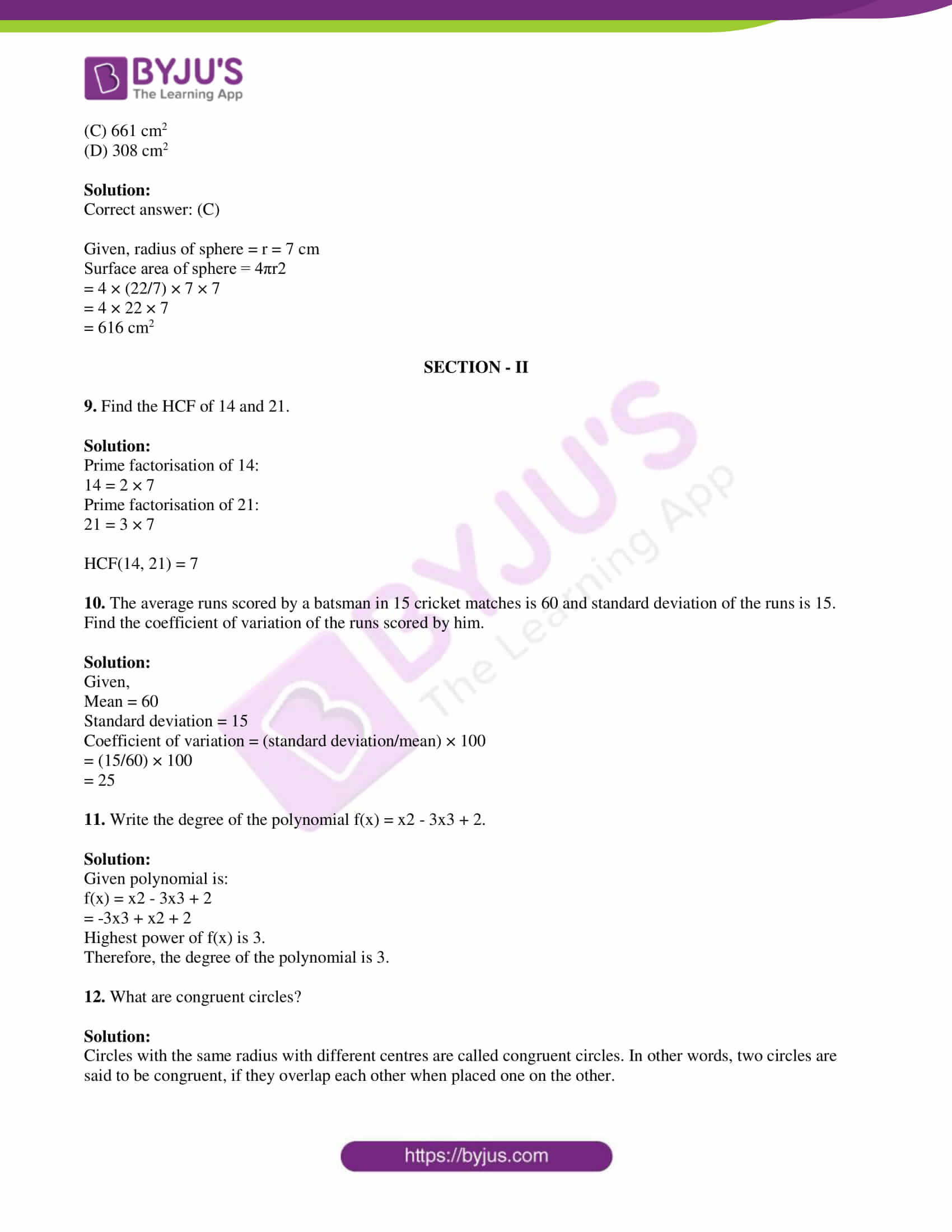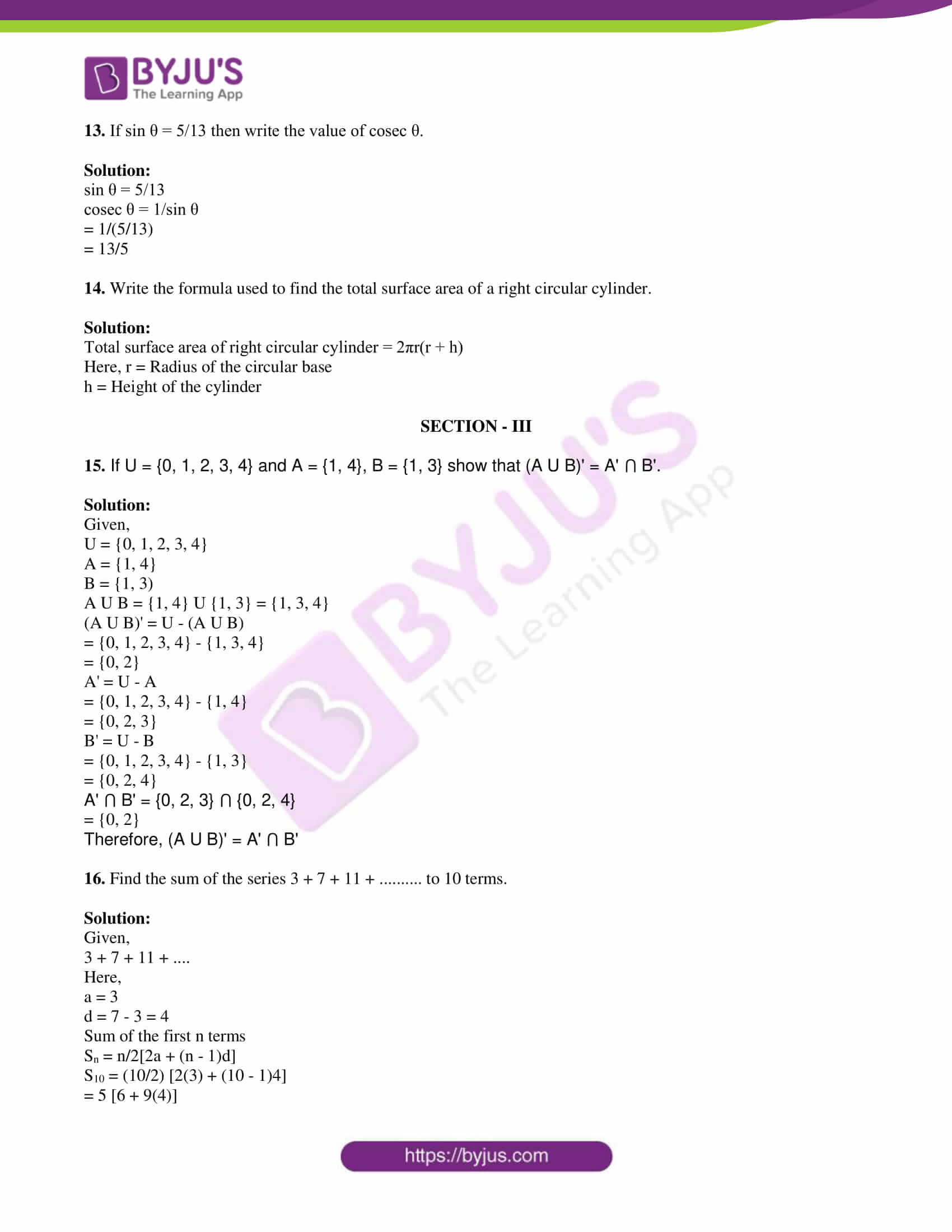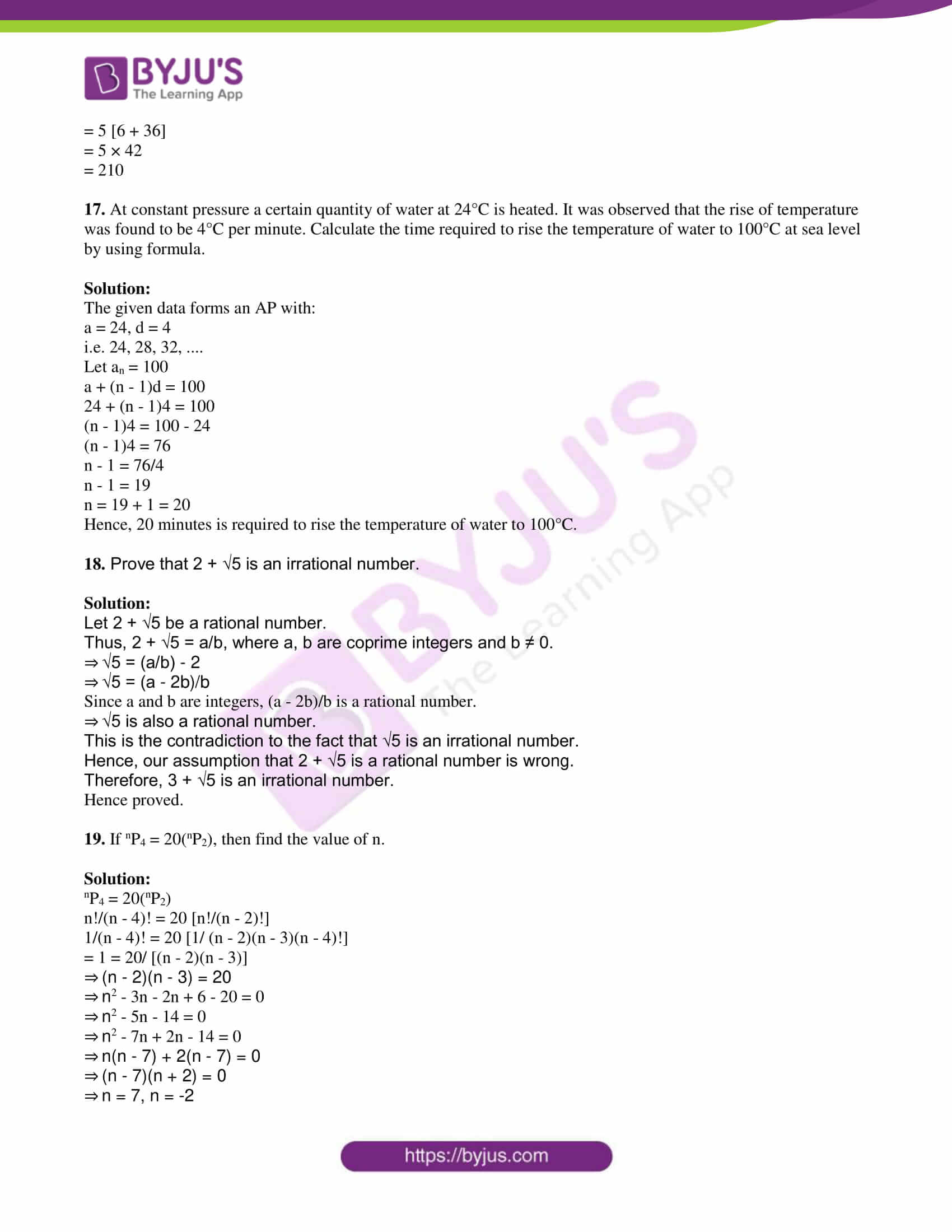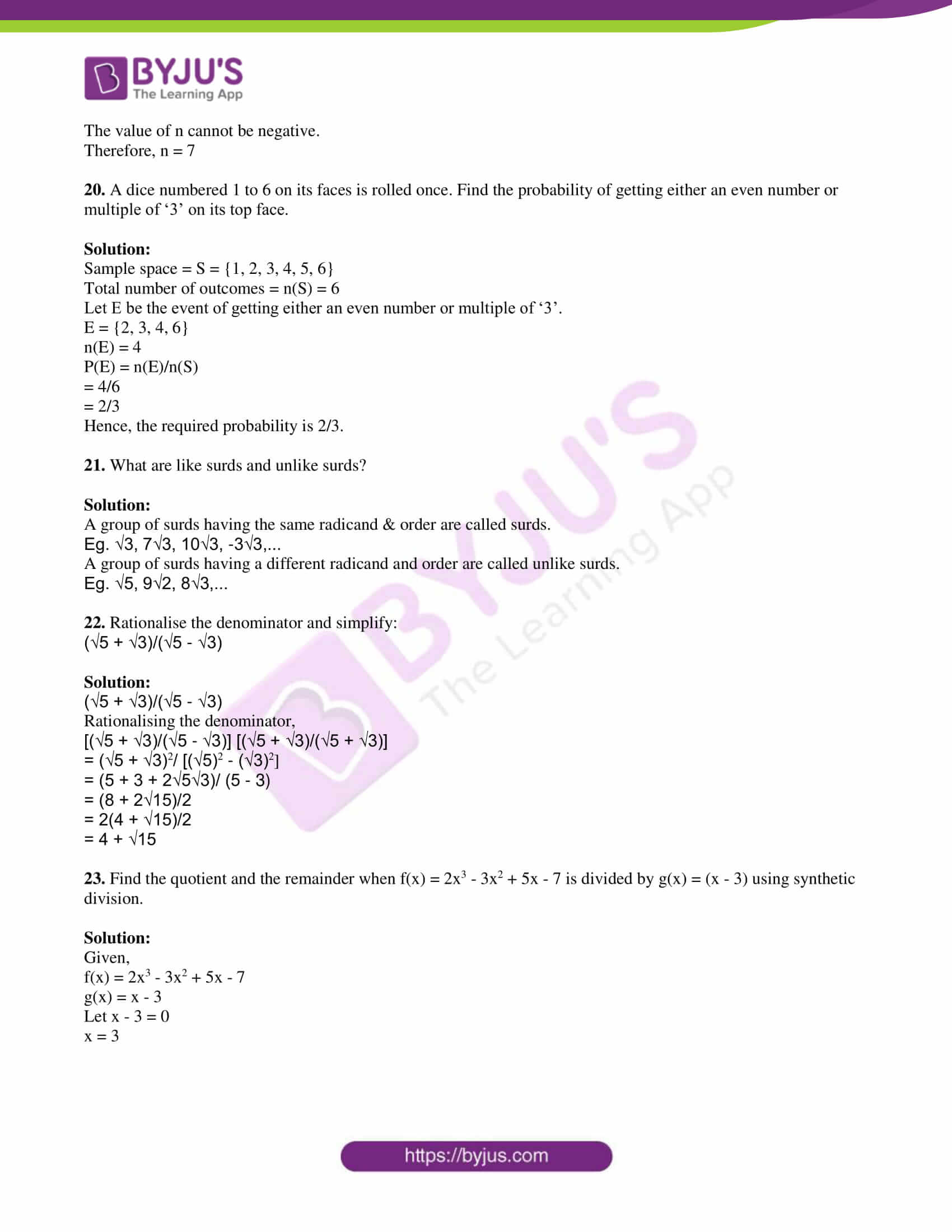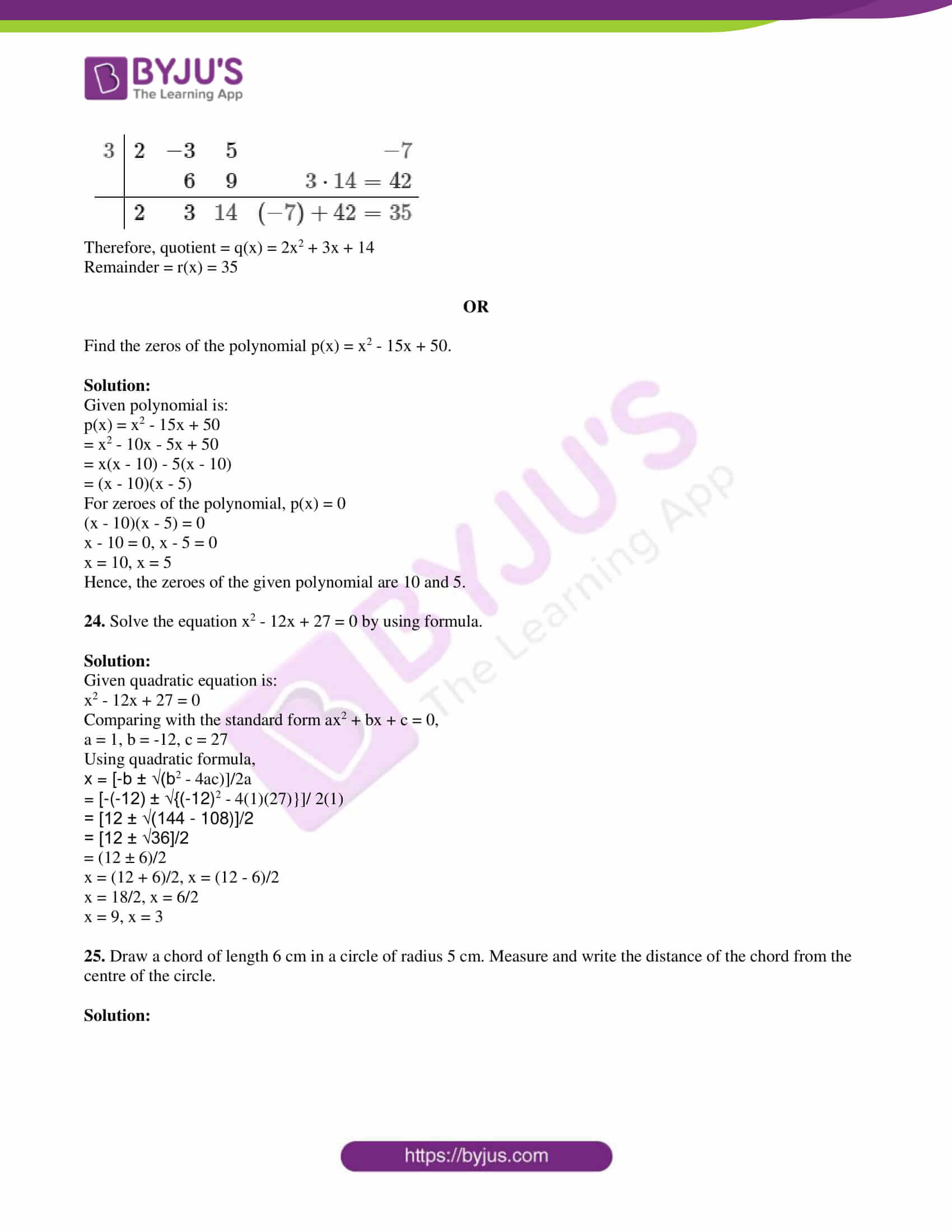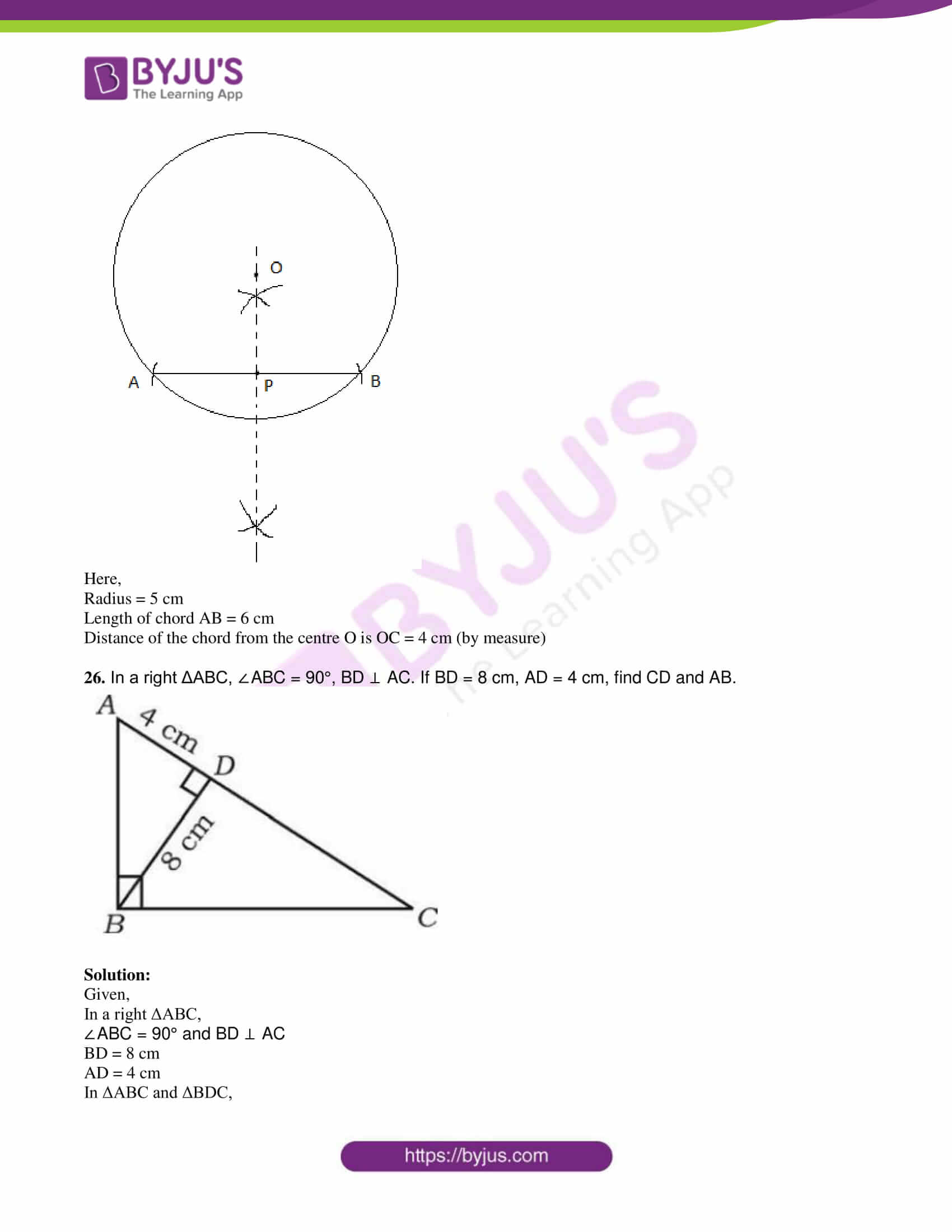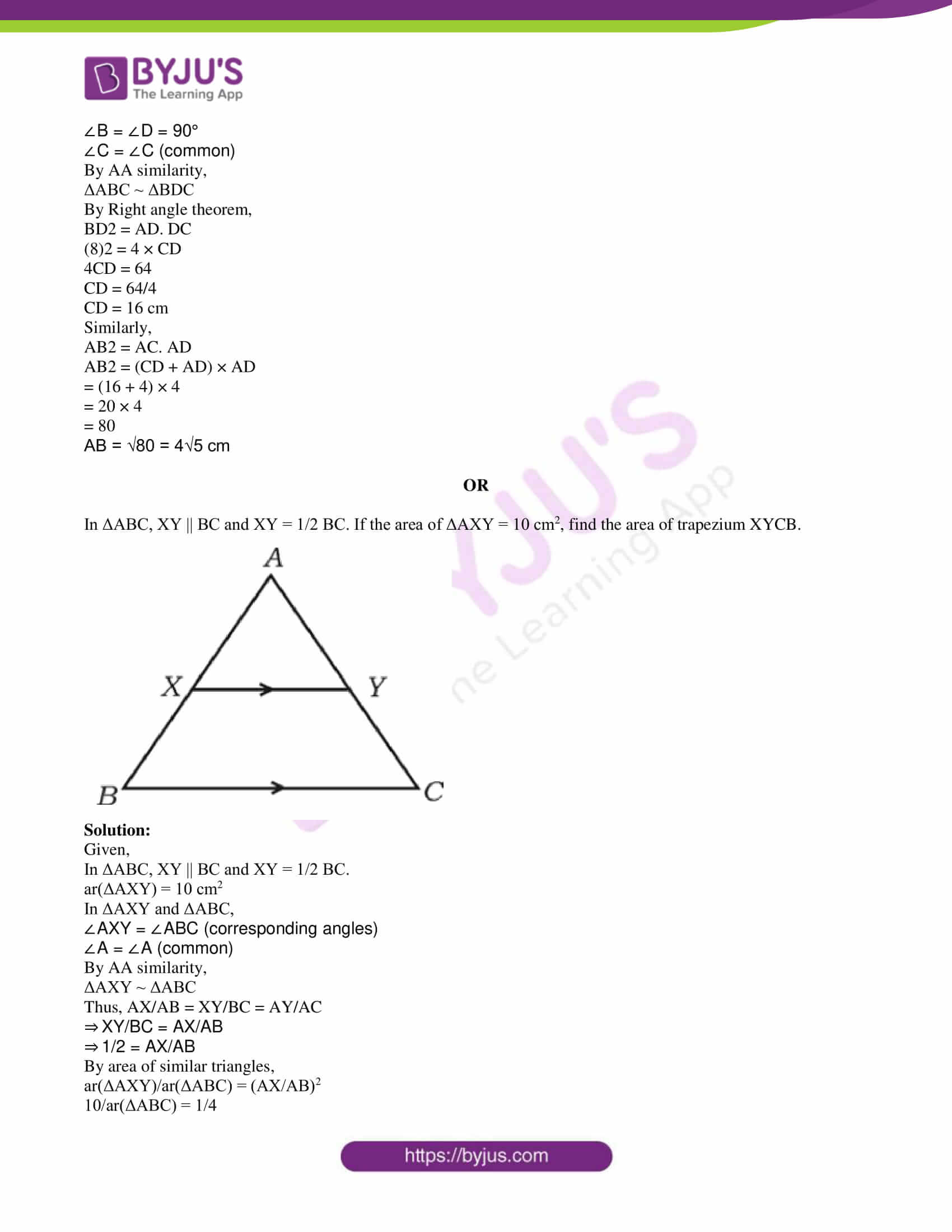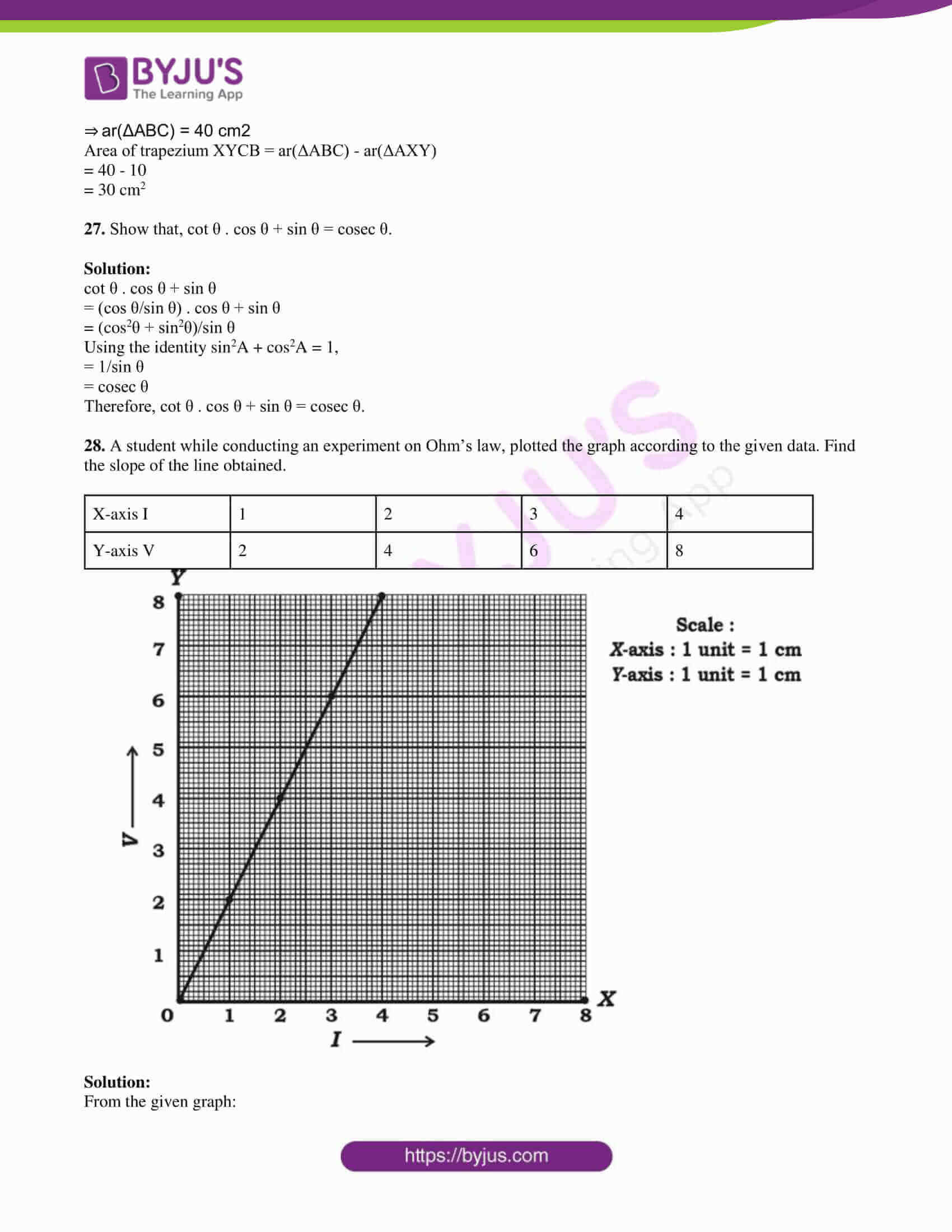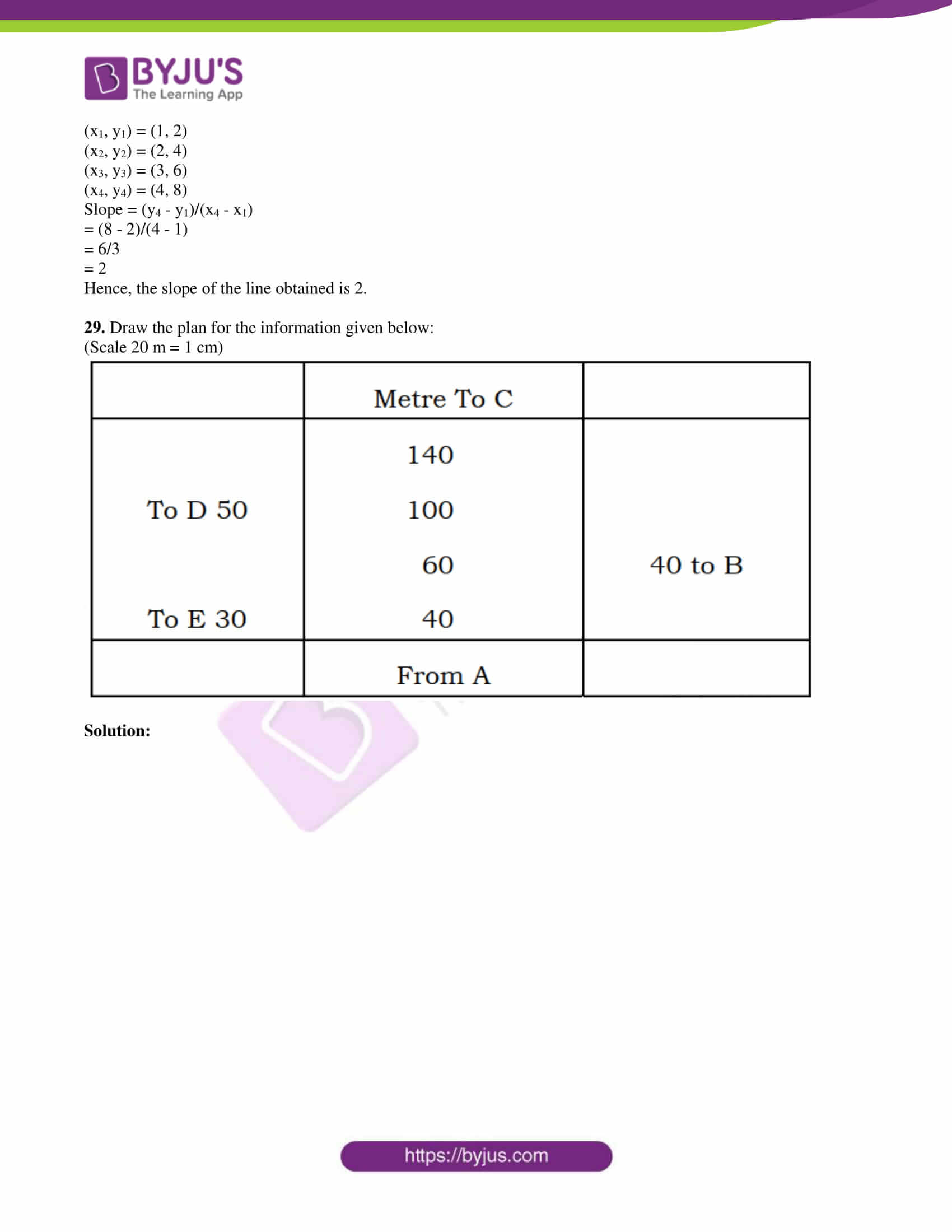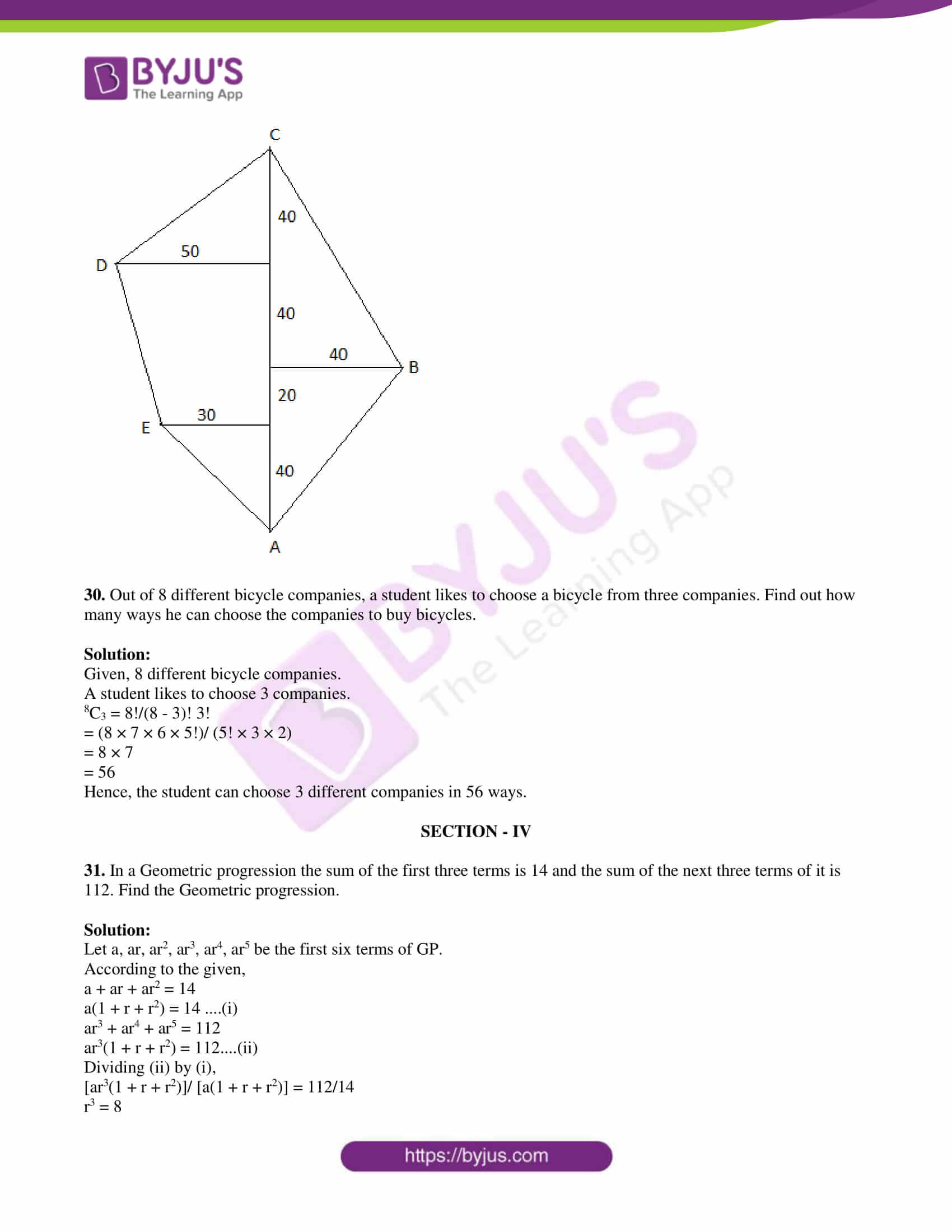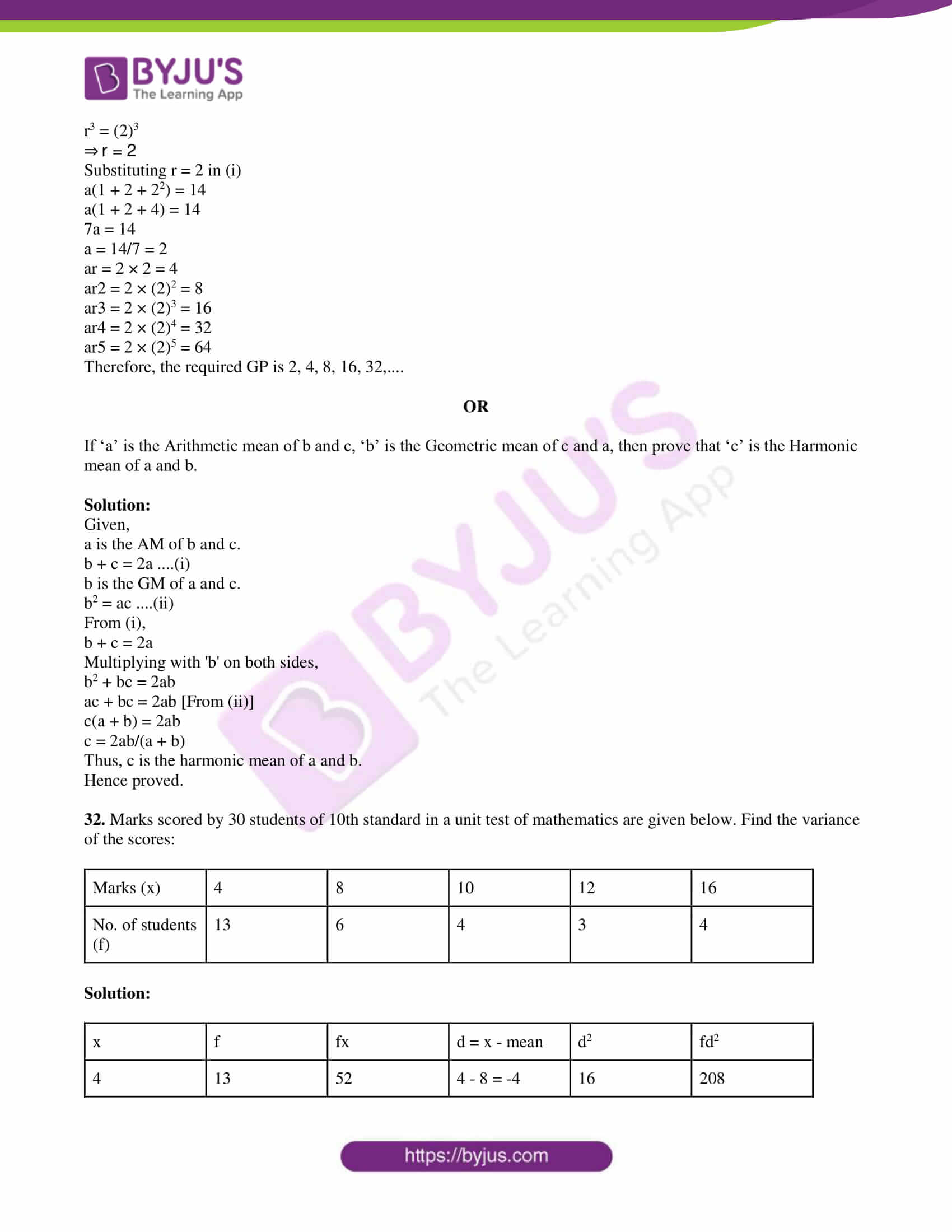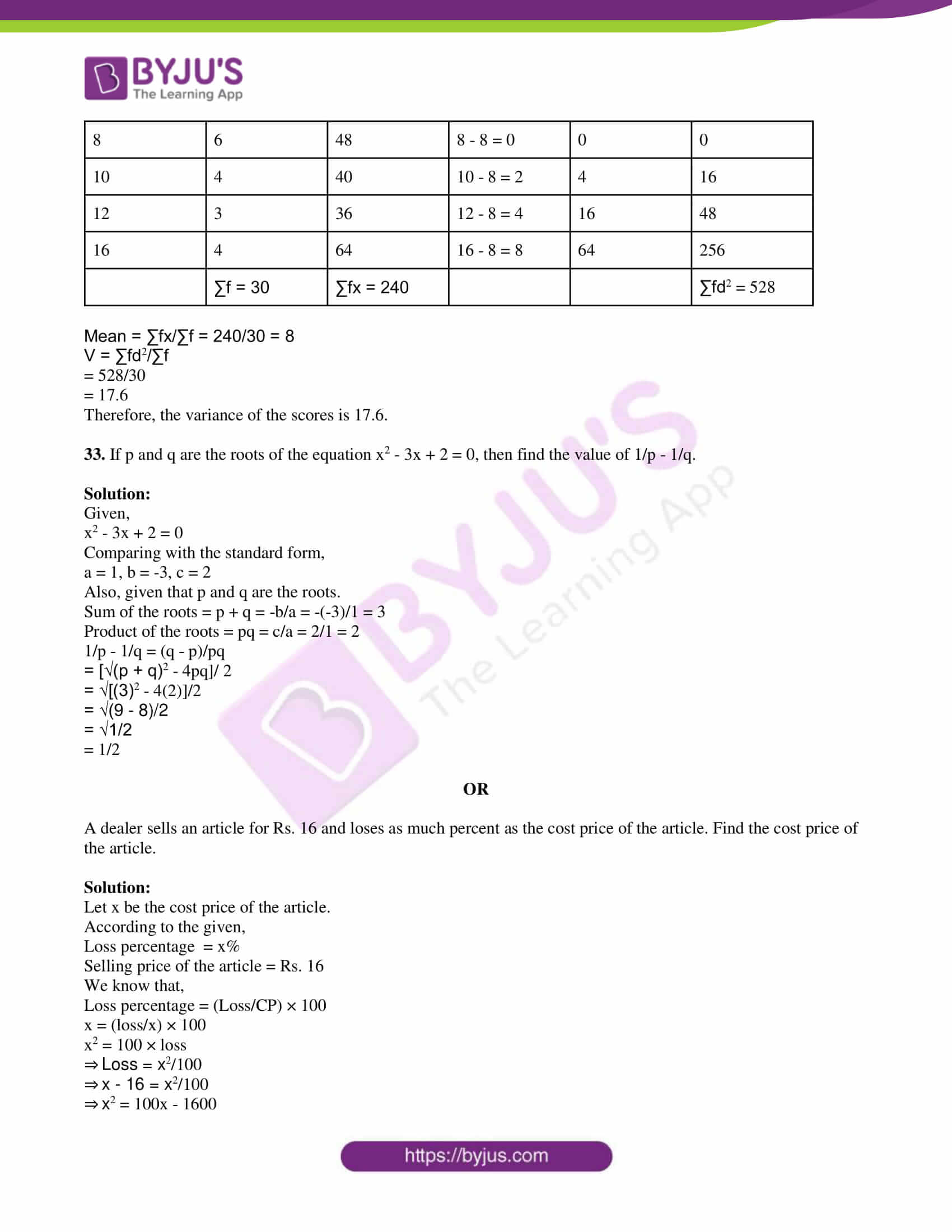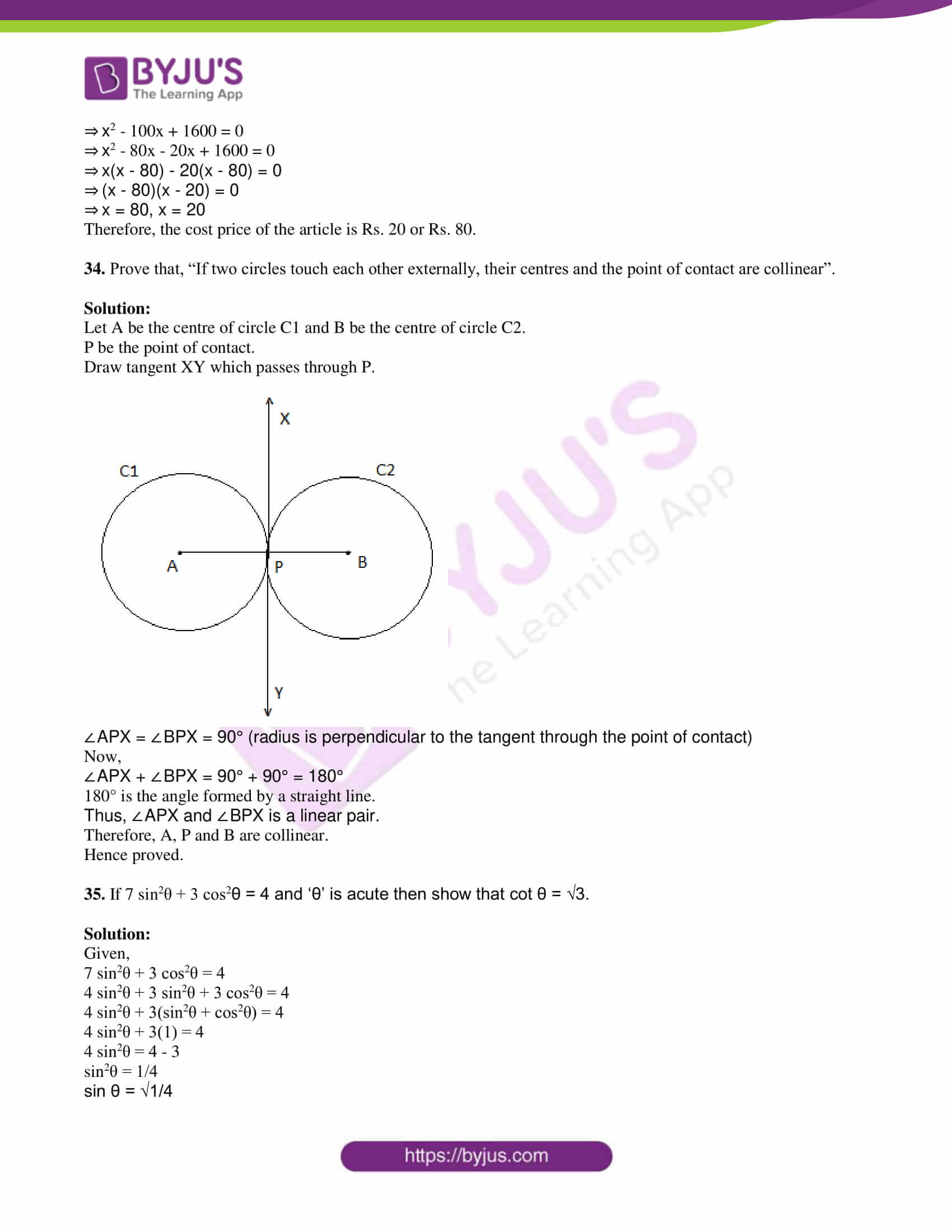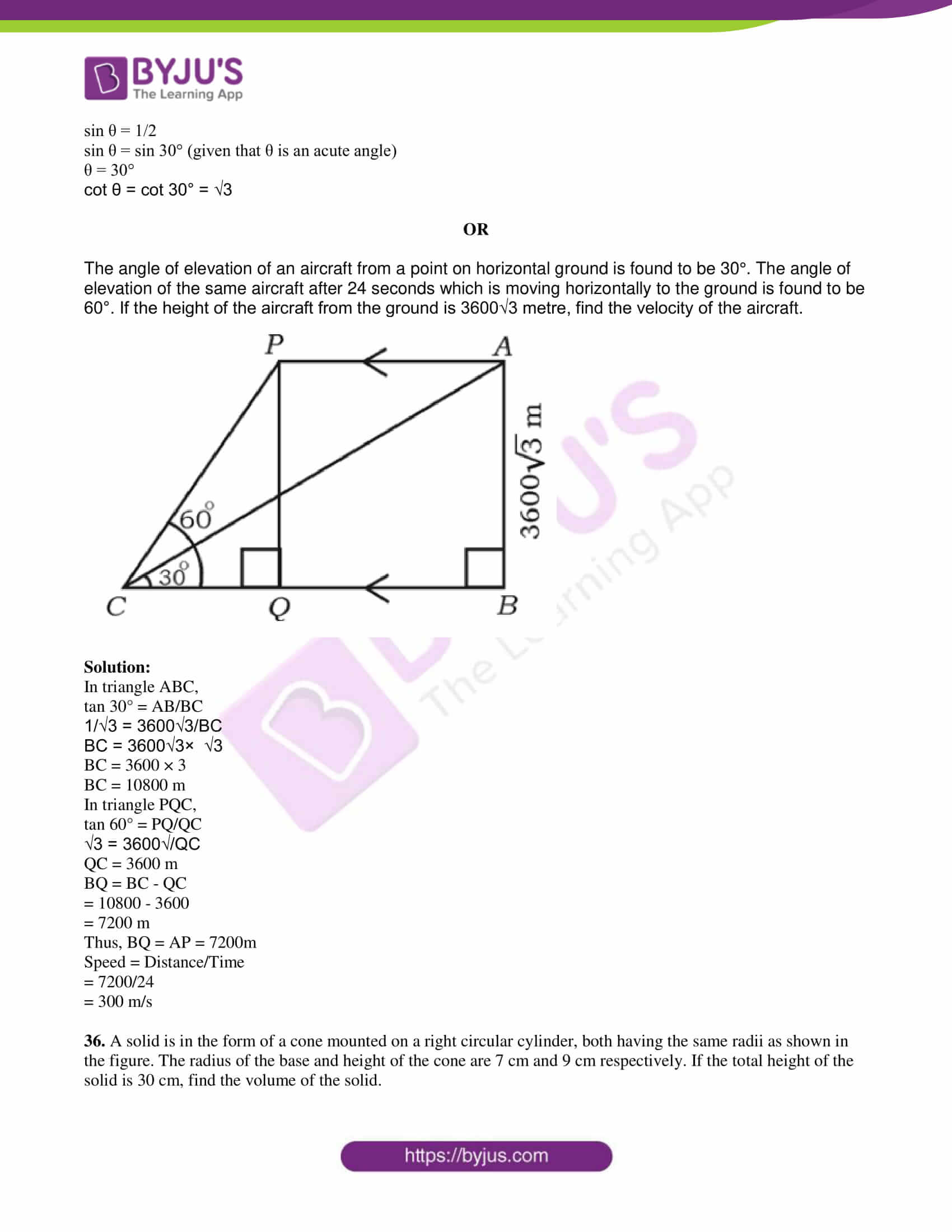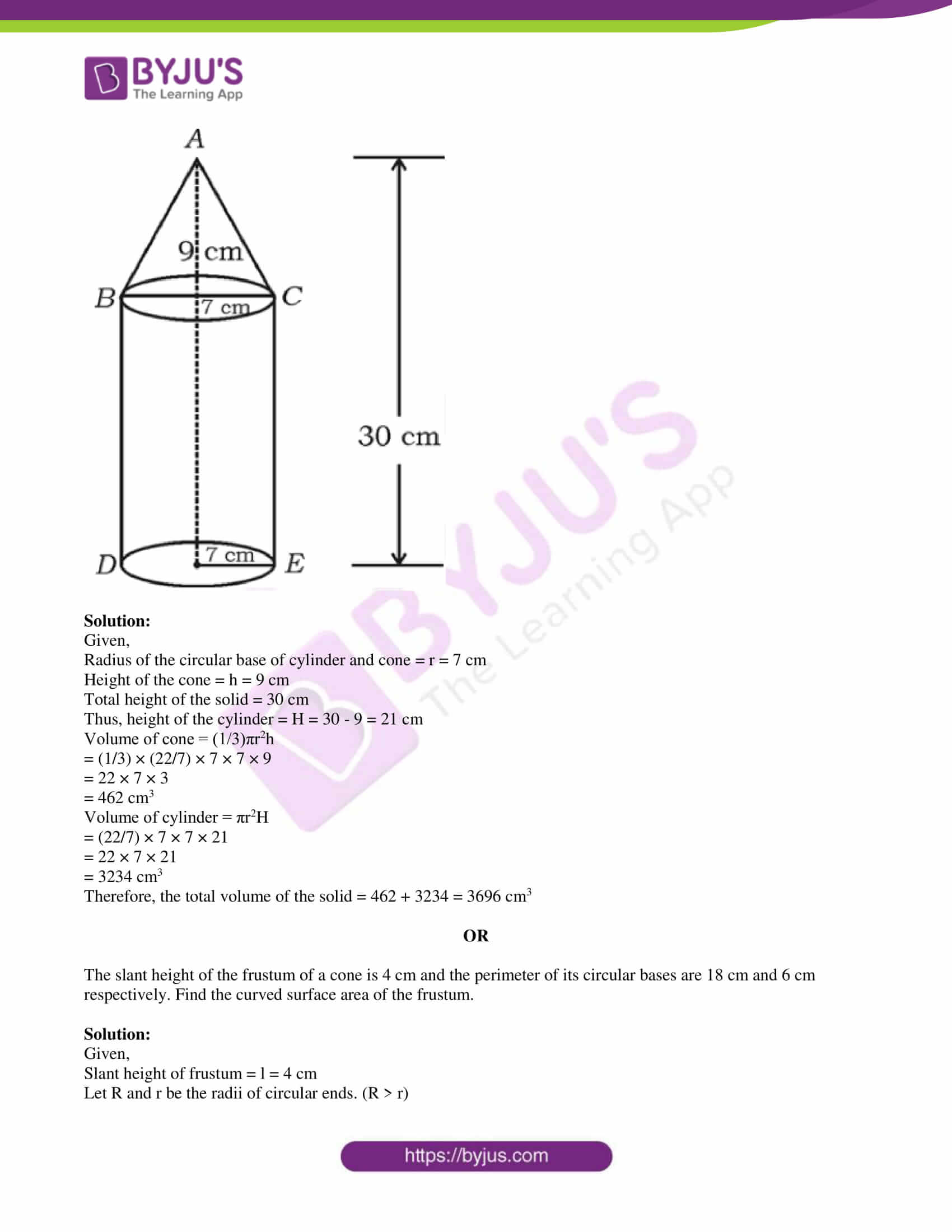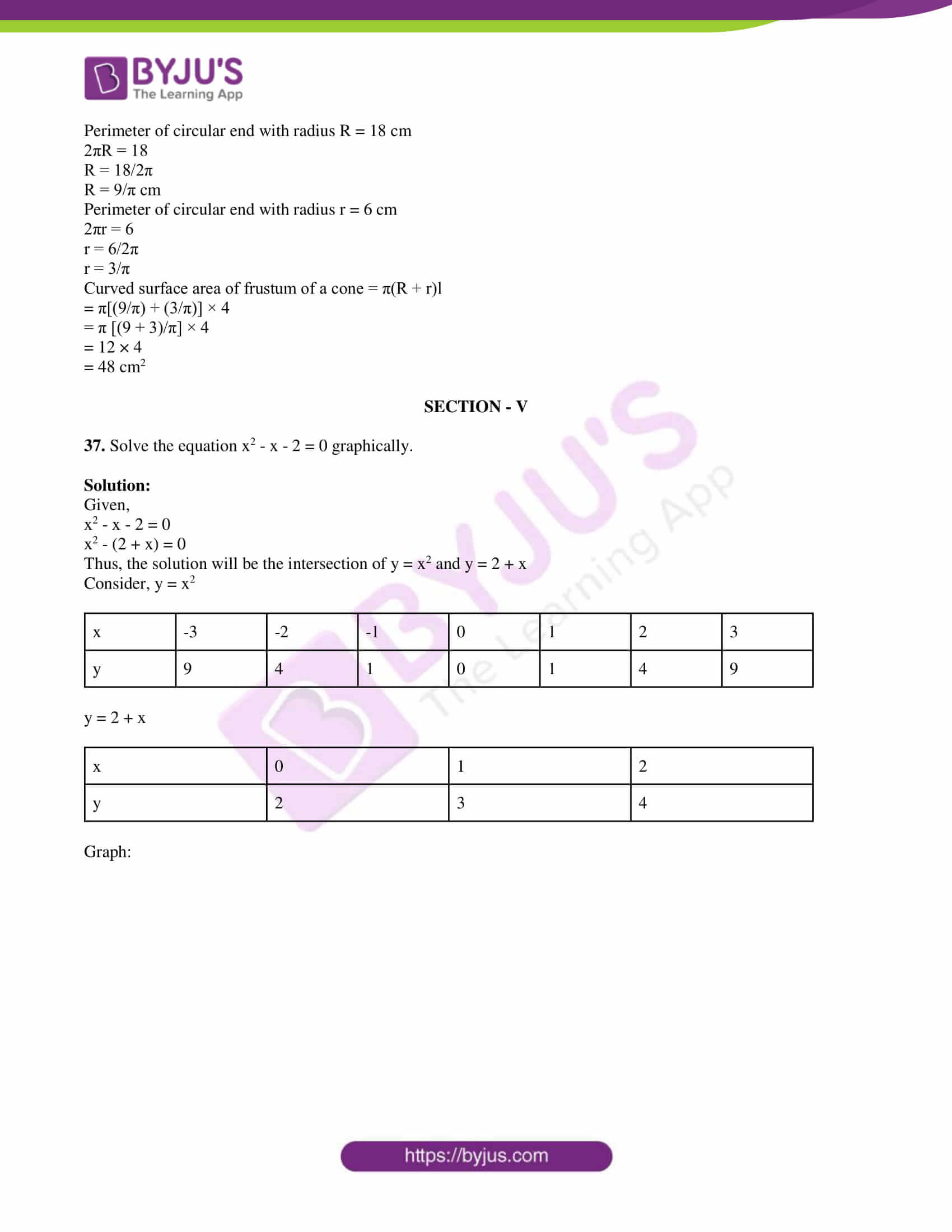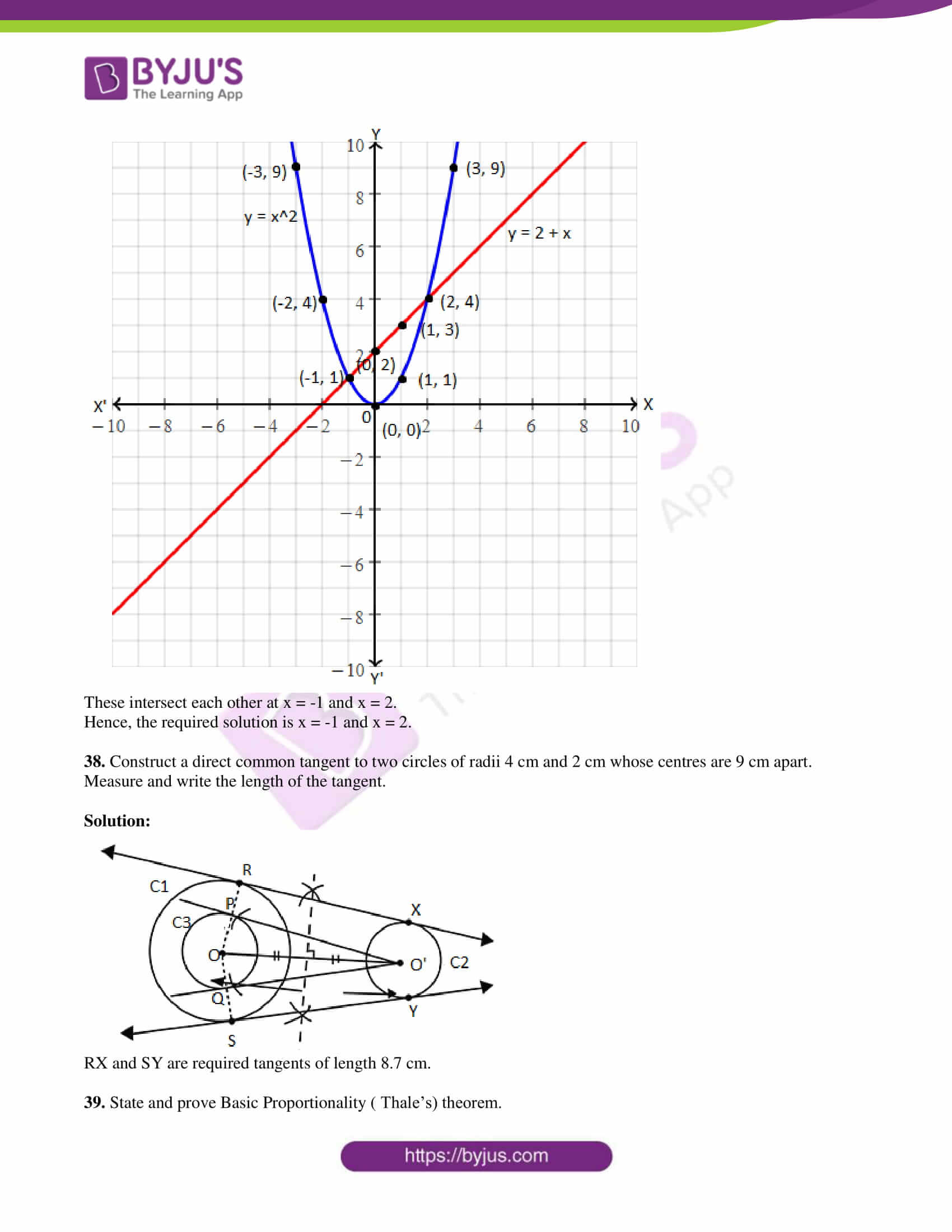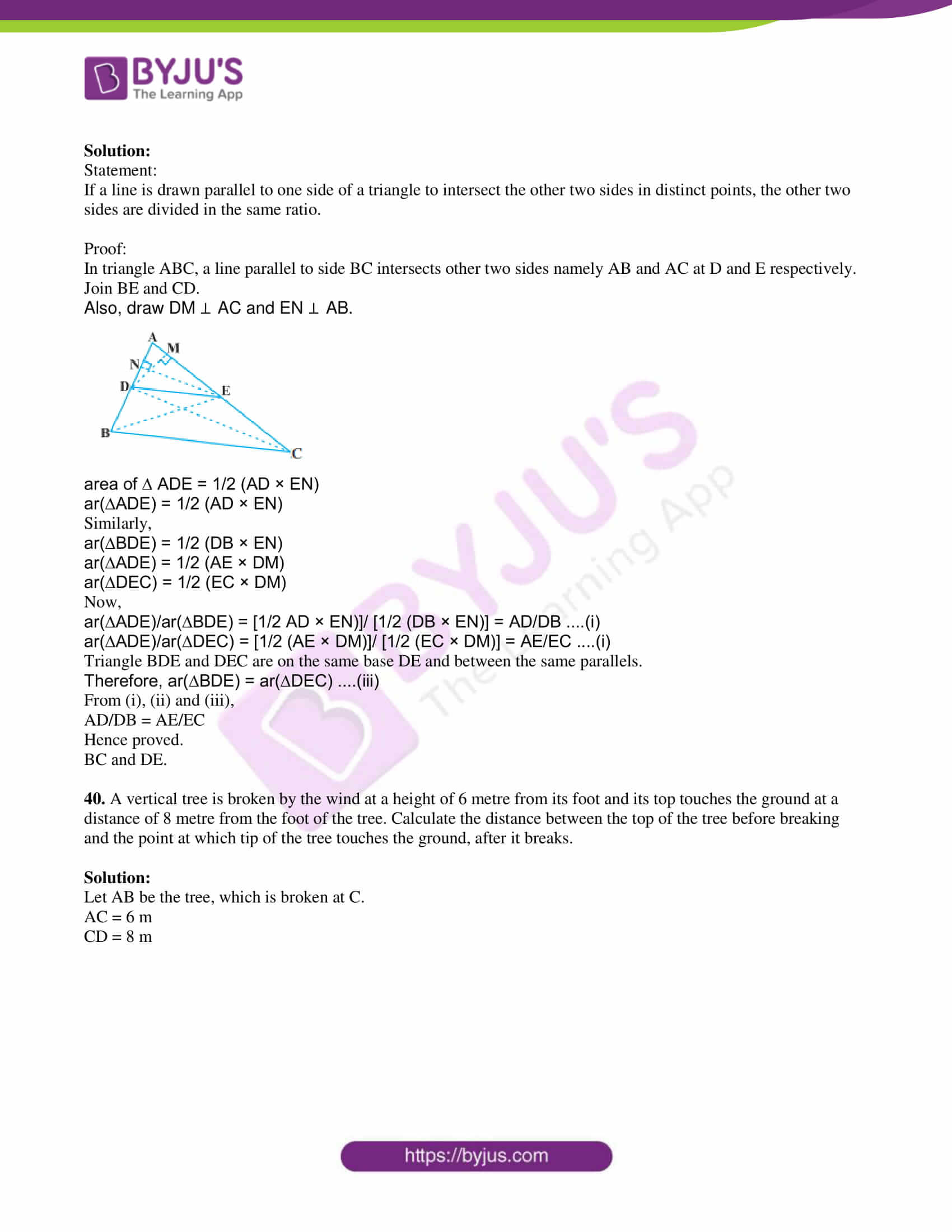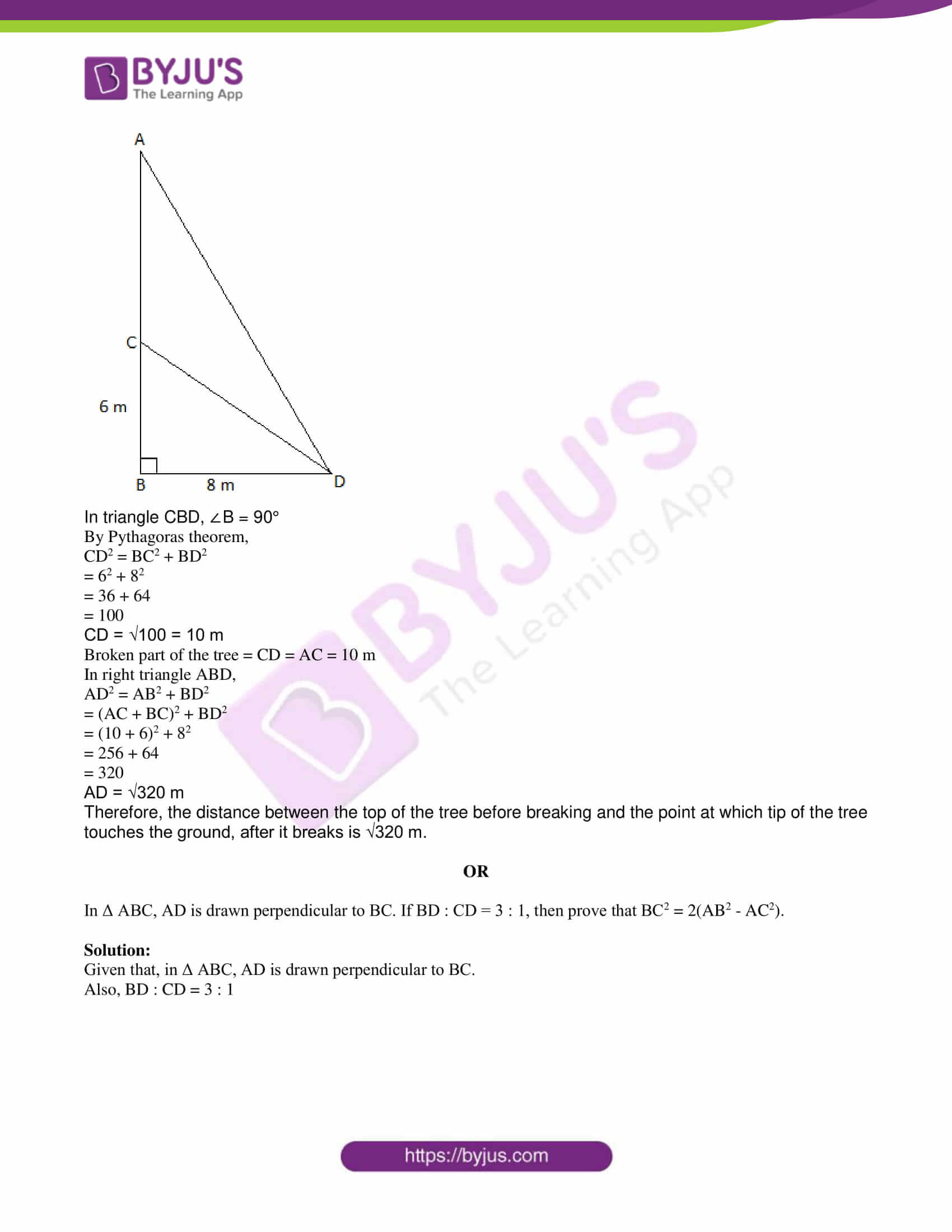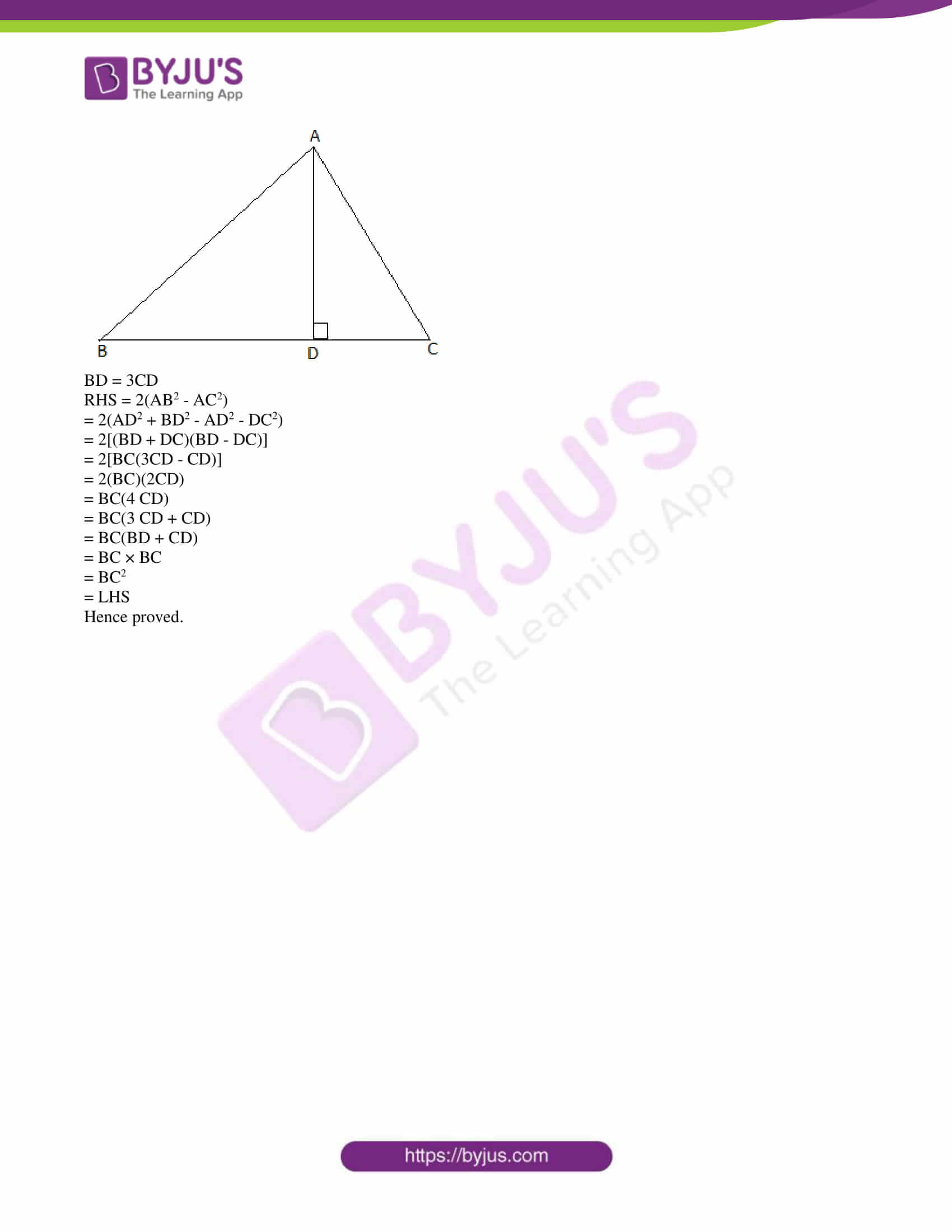## QUESTION PAPER CODE 81-E

SECTION – I

1. In the given Venn diagram n(A) is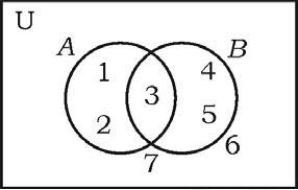(A) 3

(B) 1

(C) 5

(D) 2

Solution:

From the given figure,

The number of elements of circle A = 3

Therefore, n(A) = 3

2. Sum of all the first ‘n’ terms of even natural number is

(A) n(n + 1)

(B) n(n + 2)

(C) n2

(D) 2n2

Solution:

Even natural numbers are:

2, 4, 6, 8, …..

This is an AP with a = 2 and d = 2

Sum of the first n terms

Sn = n/2 [2a + (n – 1)d]

= n/2 [2(2) + (n – 1)2]

= n/2 [4 + 2n – 2]

= n/2 [2n + 2]

= 2n(n + 1)/2

= n(n + 1)

3. A boy has 3 shirts and 2 coats. How many different pairs, a shirt and a coat can he dress up with?

(A) 3

(B) 18

(C) 6

(D) 5

Solution:

Given,

3 shirts and 2 coats

Hence, the number of ways in which the boy can dress up with a shirt-and-coat outfit = 3 × 2 = 6

4. In a random experiment, if the occurrence of one event prevents the occurrence of other event, it is

(A) a complementary event

(B) a certain event

(C) not mutually exclusive event

(D) mutually exclusive event

Solution:

Two events are called mutually exclusive if both the events cannot occur at the same time; that means occurrence of one event prevents the occurrence of another event.

5. If the polynomial p(x) = x2 – x + 1 is divided by (x – 2), then the remainder is

(A) 2

(B) 3

(C) 0

(D) 1

Solution:

Given,

p(x) = x2 – x + 1

Let g(x) = x – 2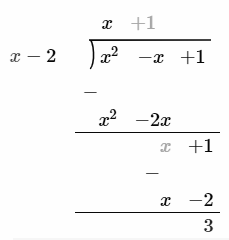Here,

Remainder = 3

6. The distance between the coordinates of a point (p, q) from the origin is

(A) p2 – q2

(B) √p2 – q2

(C) √p2 + q2

(D) q2 – p2

Solution:

Given,

Point = A(p, q)

Let O(0, 0) be the origin.

Using distance formula,

OA = √[(p – 0)2 + (q – 0)2]

= √p2 + q2

7. The equation of a line having slope 3 and y-intercept 5 is

(A) 3y = 5x + 3

(B) 5y = 3x + 5

(C) y = 3x – 5

(D) y = 3x + 5

Solution:

The equation of a line with slope m and y-intercept b is y = mx + b.

Given,

Slope = m = 3

y-intercept = b = 5

Therefore, the equation of a line is y = 3x + 5

8. The surface area of a sphere of radius 7 cm is

(A) 88 cm2

(B) 616 cm2

(C) 661 cm2

(D) 308 cm2

Solution:

Given, radius of sphere = r = 7 cm

Surface area of sphere = 4πr2

= 4 × (22/7) × 7 × 7

= 4 × 22 × 7

= 616 cm2

SECTION – II

9. Find the HCF of 14 and 21.

Solution:

Prime factorisation of 14:

14 = 2 × 7

Prime factorisation of 21:

21 = 3 × 7

HCF(14, 21) = 7

10. The average runs scored by a batsman in 15 cricket matches is 60 and standard deviation of the runs is 15. Find the coefficient of variation of the runs scored by him.

Solution:

Given,

Mean = 60

Standard deviation = 15

Coefficient of variation = (standard deviation/mean) × 100

= (15/60) × 100

= 25

11. Write the degree of the polynomial f(x) = x2 – 3×3 + 2.

Solution:

Given polynomial is:

f(x) = x2 – 3×3 + 2

= -3×3 + x2 + 2

Highest power of f(x) is 3.

Therefore, the degree of the polynomial is 3.

12. What are congruent circles?

Solution:

Circles with the same radius with different centres are called congruent circles. In other words, two circles are said to be congruent, if they overlap each other when placed one on the other.

13. If sin θ = 5/13 then write the value of cosec θ.

Solution:

sin θ = 5/13

cosec θ = 1/sin θ

= 1/(5/13)

= 13/5

14. Write the formula used to find the total surface area of a right circular cylinder.

Solution:

Total surface area of right circular cylinder = 2πr(r + h)

Here, r = Radius of the circular base

h = Height of the cylinder

SECTION – III

15. If U = {0, 1, 2, 3, 4} and A = {1, 4}, B = {1, 3} show that (A U B)’ = A’ ⋂ B’.

Solution:

Given,

U = {0, 1, 2, 3, 4}

A = {1, 4}

B = {1, 3)

A U B = {1, 4} U {1, 3} = {1, 3, 4}

(A U B)’ = U – (A U B)

= {0, 1, 2, 3, 4} – {1, 3, 4}

= {0, 2}

A’ = U – A

= {0, 1, 2, 3, 4} – {1, 4}

= {0, 2, 3}

B’ = U – B

= {0, 1, 2, 3, 4} – {1, 3}

= {0, 2, 4}

A’ ⋂ B’ = {0, 2, 3} ⋂ {0, 2, 4}

= {0, 2}

Therefore, (A U B)’ = A’ ⋂ B’

16. Find the sum of the series 3 + 7 + 11 + ………. to 10 terms.

Solution:

Given,

3 + 7 + 11 + ….

Here,

a = 3

d = 7 – 3 = 4

Sum of the first n terms

Sn = n/2[2a + (n – 1)d]

S10 = (10/2) [2(3) + (10 – 1)4]

= 5 [6 + 9(4)]

= 5 [6 + 36]

= 5 × 42

= 210

17. At constant pressure a certain quantity of water at 24°C is heated. It was observed that the rise of temperature was found to be 4°C per minute. Calculate the time required to rise the temperature of water to 100°C at sea level by using formula.

Solution:

The given data forms an AP with:

a = 24, d = 4

i.e. 24, 28, 32, ….

Let an = 100

a + (n – 1)d = 100

24 + (n – 1)4 = 100

(n – 1)4 = 100 – 24

(n – 1)4 = 76

n – 1 = 76/4

n – 1 = 19

n = 19 + 1 = 20

Hence, 20 minutes is required to rise the temperature of water to 100°C.

18. Prove that 2 + √5 is an irrational number.

Solution:

Let 2 + √5 be a rational number.

Thus, 2 + √5 = a/b, where a, b are coprime integers and b ≠ 0.

⇒ √5 = (a/b) – 2

⇒ √5 = (a – 2b)/b

Since a and b are integers, (a – 2b)/b is a rational number.

⇒ √5 is also a rational number.

This is the contradiction to the fact that √5 is an irrational number.

Hence, our assumption that 2 + √5 is a rational number is wrong.

Therefore, 3 + √5 is an irrational number.

Hence proved.

19. If nP4 = 20(nP2), then find the value of n.

Solution:

nP4 = 20(nP2)

n!/(n – 4)! = 20 [n!/(n – 2)!]

1/(n – 4)! = 20 [1/ (n – 2)(n – 3)(n – 4)!]

= 1 = 20/ [(n – 2)(n – 3)]

⇒ (n – 2)(n – 3) = 20

⇒ n2 – 3n – 2n + 6 – 20 = 0

⇒ n2 – 5n – 14 = 0

⇒ n2 – 7n + 2n – 14 = 0

⇒ n(n – 7) + 2(n – 7) = 0

⇒ (n – 7)(n + 2) = 0

⇒ n = 7, n = -2

The value of n cannot be negative.

Therefore, n = 7

20. A dice numbered 1 to 6 on its faces is rolled once. Find the probability of getting either an even number or multiple of ‘3’ on its top face.

Solution:

Sample space = S = {1, 2, 3, 4, 5, 6}

Total number of outcomes = n(S) = 6

Let E be the event of getting either an even number or multiple of ‘3’.

E = {2, 3, 4, 6}

n(E) = 4

P(E) = n(E)/n(S)

= 4/6

= 2/3

Hence, the required probability is 2/3.

21. What are like surds and unlike surds?

Solution:

A group of surds having the same radicand & order are called surds.

Eg. √3, 7√3, 10√3, -3√3,…

A group of surds having a different radicand and order are called unlike surds.

Eg. √5, 9√2, 8√3,…

22. Rationalise the denominator and simplify:

(√5 + √3)/(√5 – √3)

Solution:

(√5 + √3)/(√5 – √3)

Rationalising the denominator,

[(√5 + √3)/(√5 – √3)] [(√5 + √3)/(√5 + √3)]

= (√5 + √3)2/ [(√5)2 – (√3)2]

= (5 + 3 + 2√5√3)/ (5 – 3)

= (8 + 2√15)/2

= 2(4 + √15)/2

= 4 + √15

23. Find the quotient and the remainder when f(x) = 2x3 – 3x2 + 5x – 7 is divided by g(x) = (x – 3) using synthetic division.

Solution:

Given,

f(x) = 2x3 – 3x2 + 5x – 7

g(x) = x – 3

Let x – 3 = 0

x = 3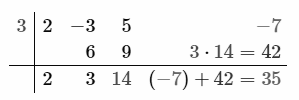Therefore, quotient = q(x) = 2x2 + 3x + 14

Remainder = r(x) = 35

OR

Find the zeros of the polynomial p(x) = x2 – 15x + 50.

Solution:

Given polynomial is:

p(x) = x2 – 15x + 50

= x2 – 10x – 5x + 50

= x(x – 10) – 5(x – 10)

= (x – 10)(x – 5)

For zeroes of the polynomial, p(x) = 0

(x – 10)(x – 5) = 0

x – 10 = 0, x – 5 = 0

x = 10, x = 5

Hence, the zeroes of the given polynomial are 10 and 5.

24. Solve the equation x2 – 12x + 27 = 0 by using formula.

Solution:

x2 – 12x + 27 = 0

Comparing with the standard form ax2 + bx + c = 0,

a = 1, b = -12, c = 27

x = [-b ± √(b2 – 4ac)]/2a

= [-(-12) ± √{(-12)2 – 4(1)(27)}]/ 2(1)

= [12 ± √(144 – 108)]/2

= [12 ± √36]/2

= (12 ± 6)/2

x = (12 + 6)/2, x = (12 – 6)/2

x = 18/2, x = 6/2

x = 9, x = 3

25. Draw a chord of length 6 cm in a circle of radius 5 cm. Measure and write the distance of the chord from the centre of the circle.

Solution: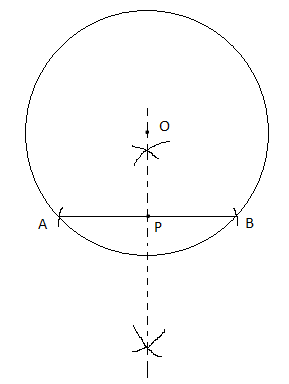Here,

Length of chord AB = 6 cm

Distance of the chord from the centre O is OC = 4 cm (by measure)

26. In a right ΔABC, ∠ABC = 90°, BD ⊥ AC. If BD = 8 cm, AD = 4 cm, find CD and AB.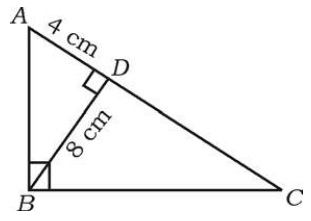Solution:

Given,

In a right ΔABC,

∠ABC = 90° and BD ⊥ AC

BD = 8 cm

In ΔABC and ΔBDC,

∠B = ∠D = 90°

∠C = ∠C (common)

By AA similarity,

ΔABC ~ ΔBDC

By Right angle theorem,

(8)2 = 4 × CD

4CD = 64

CD = 64/4

CD = 16 cm

Similarly,

= (16 + 4) × 4

= 20 × 4

= 80

AB = √80 = 4√5 cm

OR

In ΔABC, XY || BC and XY = 1/2 BC. If the area of ΔAXY = 10 cm2, find the area of trapezium XYCB.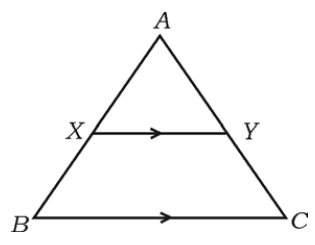Solution:

Given,

In ΔABC, XY || BC and XY = 1/2 BC.

ar(ΔAXY) = 10 cm2

In ΔAXY and ΔABC,

∠AXY = ∠ABC (corresponding angles)

∠A = ∠A (common)

By AA similarity,

ΔAXY ~ ΔABC

Thus, AX/AB = XY/BC = AY/AC

⇒ XY/BC = AX/AB

⇒ 1/2 = AX/AB

By area of similar triangles,

ar(ΔAXY)/ar(ΔABC) = (AX/AB)2

10/ar(ΔABC) = 1/4

⇒ ar(ΔABC) = 40 cm2

Area of trapezium XYCB = ar(ΔABC) – ar(ΔAXY)

= 40 – 10

= 30 cm2

27. Show that, cot θ . cos θ + sin θ = cosec θ.

Solution:

cot θ . cos θ + sin θ

= (cos θ/sin θ) . cos θ + sin θ

= (cos2θ + sin2θ)/sin θ

Using the identity sin2A + cos2A = 1,

= 1/sin θ

= cosec θ

Therefore, cot θ . cos θ + sin θ = cosec θ.

28. A student while conducting an experiment on Ohm’s law, plotted the graph according to the given data. Find the slope of the line obtained.

 X-axis I 1 2 3 4 Y-axis V 2 4 6 8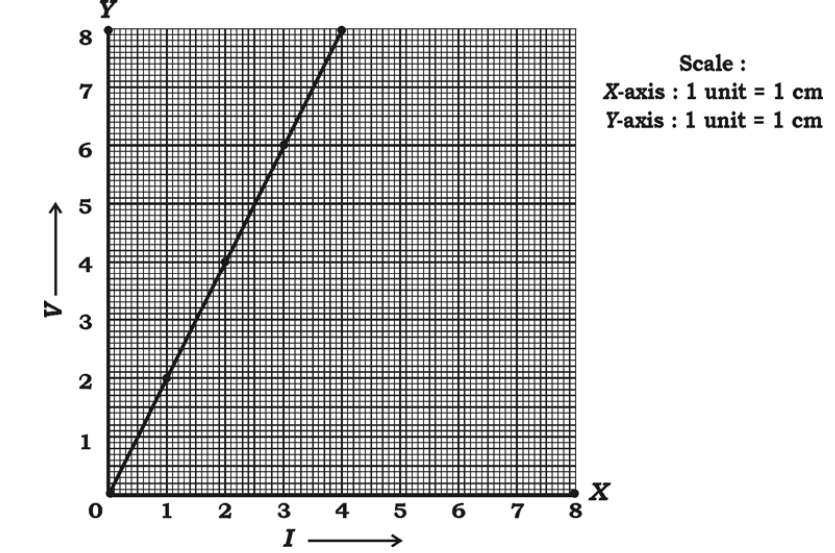Solution:

From the given graph:

(x1, y1) = (1, 2)

(x2, y2) = (2, 4)

(x3, y3) = (3, 6)

(x4, y4) = (4, 8)

Slope = (y4 – y1)/(x4 – x1)

= (8 – 2)/(4 – 1)

= 6/3

= 2

Hence, the slope of the line obtained is 2.

29. Draw the plan for the information given below:

(Scale 20 m = 1 cm)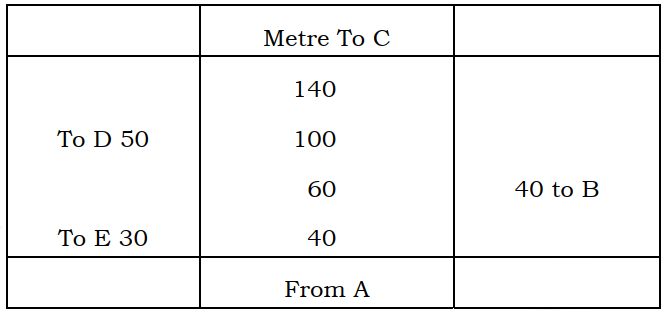Solution: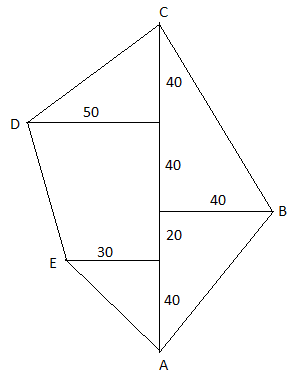30. Out of 8 different bicycle companies, a student likes to choose a bicycle from three companies. Find out how many ways he can choose the companies to buy bicycles.

Solution:

Given, 8 different bicycle companies.

A student likes to choose 3 companies.

8C3 = 8!/(8 – 3)! 3!

= (8 × 7 × 6 × 5!)/ (5! × 3 × 2)

= 8 × 7

= 56

Hence, the student can choose 3 different companies in 56 ways.

SECTION – IV

31. In a Geometric progression the sum of the first three terms is 14 and the sum of the next three terms of it is 112. Find the Geometric progression.

Solution:

Let a, ar, ar2, ar3, ar4, ar5 be the first six terms of GP.

According to the given,

a + ar + ar2 = 14

a(1 + r + r2) = 14 ….(i)

ar3 + ar4 + ar5 = 112

ar3(1 + r + r2) = 112….(ii)

Dividing (ii) by (i),

[ar3(1 + r + r2)]/ [a(1 + r + r2)] = 112/14

r3 = 8

r3 = (2)3

⇒ r = 2

Substituting r = 2 in (i)

a(1 + 2 + 22) = 14

a(1 + 2 + 4) = 14

7a = 14

a = 14/7 = 2

ar = 2 × 2 = 4

ar2 = 2 × (2)2 = 8

ar3 = 2 × (2)3 = 16

ar4 = 2 × (2)4 = 32

ar5 = 2 × (2)5 = 64

Therefore, the required GP is 2, 4, 8, 16, 32,….

OR

If ‘a’ is the Arithmetic mean of b and c, ‘b’ is the Geometric mean of c and a, then prove that ‘c’ is the Harmonic mean of a and b.

Solution:

Given,

a is the AM of b and c.

b + c = 2a ….(i)

b is the GM of a and c.

b2 = ac ….(ii)

From (i),

b + c = 2a

Multiplying with ‘b’ on both sides,

b2 + bc = 2ab

ac + bc = 2ab [From (ii)]

c(a + b) = 2ab

c = 2ab/(a + b)

Thus, c is the harmonic mean of a and b.

Hence proved.

32. Marks scored by 30 students of 10th standard in a unit test of mathematics are given below. Find the variance of the scores:

 Marks (x) 4 8 10 12 16 No. of students (f) 13 6 4 3 4

Solution:

 x f fx d = x – mean d2 fd2 4 13 52 4 – 8 = -4 16 208 8 6 48 8 – 8 = 0 0 0 10 4 40 10 – 8 = 2 4 16 12 3 36 12 – 8 = 4 16 48 16 4 64 16 – 8 = 8 64 256 ∑f = 30 ∑fx = 240 ∑fd2 = 528

Mean = ∑fx/∑f = 240/30 = 8

V = ∑fd2/∑f

= 528/30

= 17.6

Therefore, the variance of the scores is 17.6.

33. If p and q are the roots of the equation x2 – 3x + 2 = 0, then find the value of 1/p – 1/q.

Solution:

Given,

x2 – 3x + 2 = 0

Comparing with the standard form,

a = 1, b = -3, c = 2

Also, given that p and q are the roots.

Sum of the roots = p + q = -b/a = -(-3)/1 = 3

Product of the roots = pq = c/a = 2/1 = 2

1/p – 1/q = (q – p)/pq

= [√(p + q)2 – 4pq]/ 2

= √[(3)2 – 4(2)]/2

= √(9 – 8)/2

= √1/2

= 1/2

OR

A dealer sells an article for Rs. 16 and loses as much percent as the cost price of the article. Find the cost price of the article.

Solution:

Let x be the cost price of the article.

According to the given,

Loss percentage = x%

Selling price of the article = Rs. 16

We know that,

Loss percentage = (Loss/CP) × 100

x = (loss/x) × 100

x2 = 100 × loss

⇒ Loss = x2/100

⇒ x – 16 = x2/100

⇒ x2 = 100x – 1600

⇒ x2 – 100x + 1600 = 0

⇒ x2 – 80x – 20x + 1600 = 0

⇒ x(x – 80) – 20(x – 80) = 0

⇒ (x – 80)(x – 20) = 0

⇒ x = 80, x = 20

Therefore, the cost price of the article is Rs. 20 or Rs. 80.

34. Prove that, “If two circles touch each other externally, their centres and the point of contact are collinear”.

Solution:

Let A be the centre of circle C1 and B be the centre of circle C2.

P be the point of contact.

Draw tangent XY which passes through P.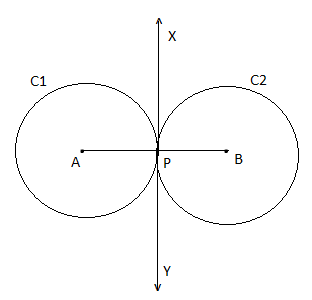∠APX = ∠BPX = 90° (radius is perpendicular to the tangent through the point of contact)

Now,

∠APX + ∠BPX = 90° + 90° = 180°

180° is the angle formed by a straight line.

Thus, ∠APX and ∠BPX is a linear pair.

Therefore, A, P and B are collinear.

Hence proved.

35. If 7 sin2θ + 3 cos2θ = 4 and ‘θ’ is acute then show that cot θ = √3.

Solution:

Given,

7 sin2θ + 3 cos2θ = 4

4 sin2θ + 3 sin2θ + 3 cos2θ = 4

4 sin2θ + 3(sin2θ + cos2θ) = 4

4 sin2θ + 3(1) = 4

4 sin2θ = 4 – 3

sin2θ = 1/4

sin θ = √1/4

sin θ = 1/2

sin θ = sin 30° (given that θ is an acute angle)

θ = 30°

cot θ = cot 30° = √3

OR

The angle of elevation of an aircraft from a point on horizontal ground is found to be 30°. The angle of elevation of the same aircraft after 24 seconds which is moving horizontally to the ground is found to be 60°. If the height of the aircraft from the ground is 3600√3 metre, find the velocity of the aircraft.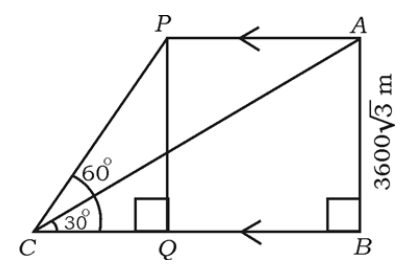Solution:

In triangle ABC,

tan 30° = AB/BC

1/√3 = 3600√3/BC

BC = 3600√3× √3

BC = 3600 × 3

BC = 10800 m

In triangle PQC,

tan 60° = PQ/QC

√3 = 3600√/QC

QC = 3600 m

BQ = BC – QC

= 10800 – 3600

= 7200 m

Thus, BQ = AP = 7200m

Speed = Distance/Time

= 7200/24

= 300 m/s

36. A solid is in the form of a cone mounted on a right circular cylinder, both having the same radii as shown in the figure. The radius of the base and height of the cone are 7 cm and 9 cm respectively. If the total height of the solid is 30 cm, find the volume of the solid.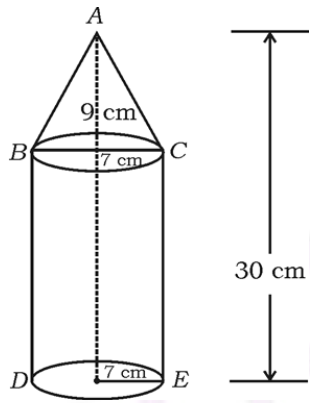Solution:

Given,

Radius of the circular base of cylinder and cone = r = 7 cm

Height of the cone = h = 9 cm

Total height of the solid = 30 cm

Thus, height of the cylinder = H = 30 – 9 = 21 cm

Volume of cone = (1/3)πr2h

= (1/3) × (22/7) × 7 × 7 × 9

= 22 × 7 × 3

= 462 cm3

Volume of cylinder = πr2H

= (22/7) × 7 × 7 × 21

= 22 × 7 × 21

= 3234 cm3

Therefore, the total volume of the solid = 462 + 3234 = 3696 cm3

OR

The slant height of the frustum of a cone is 4 cm and the perimeter of its circular bases are 18 cm and 6 cm respectively. Find the curved surface area of the frustum.

Solution:

Given,

Slant height of frustum = l = 4 cm

Let R and r be the radii of circular ends. (R > r)

Perimeter of circular end with radius R = 18 cm

2πR = 18

R = 18/2π

R = 9/π cm

Perimeter of circular end with radius r = 6 cm

2πr = 6

r = 6/2π

r = 3/π

Curved surface area of frustum of a cone = π(R + r)l

= π[(9/π) + (3/π)] × 4

= π [(9 + 3)/π] × 4

= 12 × 4

= 48 cm2

SECTION – V

37. Solve the equation x2 – x – 2 = 0 graphically.

Solution:

Given,

x2 – x – 2 = 0

x2 – (2 + x) = 0

Thus, the solution will be the intersection of y = x2 and y = 2 + x

Consider, y = x2

 x -3 -2 -1 0 1 2 3 y 9 4 1 0 1 4 9

y = 2 + x

 x 0 1 2 y 2 3 4

Graph: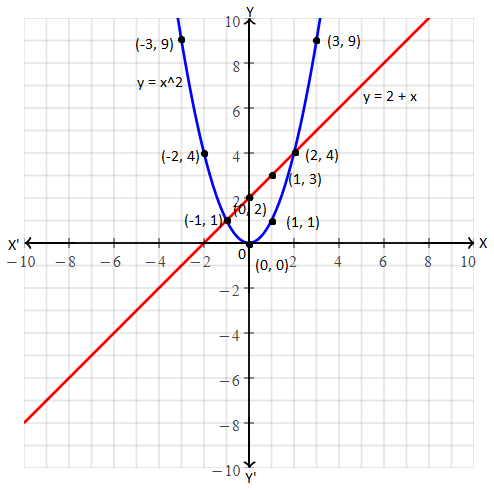These intersect each other at x = -1 and x = 2.

Hence, the required solution is x = -1 and x = 2.

38. Construct a direct common tangent to two circles of radii 4 cm and 2 cm whose centres are 9 cm apart. Measure and write the length of the tangent.

Solution: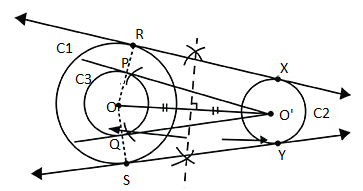RX and SY are required tangents of length 8.7 cm.

39. State and prove Basic Proportionality ( Thale’s) theorem.

Solution:

Statement:

If a line is drawn parallel to one side of a triangle to intersect the other two sides in distinct points, the other two sides are divided in the same ratio.

Proof:

In triangle ABC, a line parallel to side BC intersects other two sides namely AB and AC at D and E respectively.

Join BE and CD.

Also, draw DM ⊥ AC and EN ⊥ AB.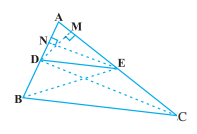Similarly,

ar(∆BDE) = 1/2 (DB × EN)

ar(∆ADE) = 1/2 (AE × DM)

ar(∆DEC) = 1/2 (EC × DM)

Now,

ar(∆ADE)/ar(∆DEC) = [1/2 (AE × DM)]/ [1/2 (EC × DM)] = AE/EC ….(i)

Triangle BDE and DEC are on the same base DE and between the same parallels.

Therefore, ar(∆BDE) = ar(∆DEC) ….(iii)

From (i), (ii) and (iii),

Hence proved.

BC and DE.

40. A vertical tree is broken by the wind at a height of 6 metre from its foot and its top touches the ground at a distance of 8 metre from the foot of the tree. Calculate the distance between the top of the tree before breaking and the point at which tip of the tree touches the ground, after it breaks.

Solution:

Let AB be the tree, which is broken at C.

AC = 6 m

CD = 8 m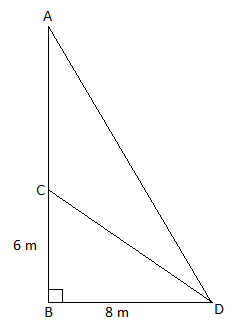In triangle CBD, ∠B = 90°

By Pythagoras theorem,

CD2 = BC2 + BD2

= 62 + 82

= 36 + 64

= 100

CD = √100 = 10 m

Broken part of the tree = CD = AC = 10 m

In right triangle ABD,

= (AC + BC)2 + BD2

= (10 + 6)2 + 82

= 256 + 64

= 320

Therefore, the distance between the top of the tree before breaking and the point at which tip of the tree touches the ground, after it breaks is √320 m.

OR

In Δ ABC, AD is drawn perpendicular to BC. If BD : CD = 3 : 1, then prove that BC2 = 2(AB2 – AC2).

Solution:

Given that, in Δ ABC, AD is drawn perpendicular to BC.

Also, BD : CD = 3 : 1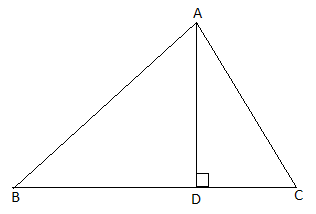BD = 3CD

RHS = 2(AB2 – AC2)

= 2[(BD + DC)(BD – DC)]

= 2[BC(3CD – CD)]

= 2(BC)(2CD)

= BC(4 CD)

= BC(3 CD + CD)

= BC(BD + CD)

= BC × BC

= BC2

= LHS

Hence proved.